# ggplot and model fitting

Plotting with ggplot and fitting statistical models

Get source code for this RMarkdown script here.

## Consider being a patron and supporting my work?

Donate and become a patron: If you find value in what I do and have learned something from my site, please consider becoming a patron. It takes me many hours to research, learn, and put together tutorials. Your support really matters.

Use `library()` to load packages at the top of each R script.

``````
library(tidyverse); library(data.table)
library(lme4); library(lmerTest); library(ggbeeswarm)
library(hausekeep)``````

## Read data from folder/directory into R

Read in data from a csv file (stored in “./data/simpsonsParadox.csv”). Right-click to download and save the data here. You can also use the `fread()` function to read and download it directly from the URL (see code below).

``````

df1``````
``````
1:  94.5128 67.9295     a
2:  95.4359 82.5449     a
3:  97.7949 69.0833     a
4:  98.1026 83.3141     a
5:  96.5641 99.0833     a
6: 101.5897 89.8526     a
7: 100.8718 73.6987     a
8:  97.0769 47.9295     a
9:  94.2051 55.6218     a
10:  94.4103 44.4679     a
11: 103.7436 74.0833     b
12: 102.8205 59.8526     b
13: 101.5897 47.9295     b
14: 105.3846 44.8526     b
15: 106.4103 60.2372     b
16: 109.4872 64.8526     b
17: 107.2308 74.4679     b
18: 107.2308 49.8526     b
19: 102.1026 37.9295     b
20: 100.0513 54.8526     b
21: 111.0256 56.0064     c
22: 114.7179 56.0064     c
23: 112.2564 46.3910     c
24: 108.6667 43.6987     c
25: 110.5128 36.3910     c
26: 114.1026 30.2372     c
27: 115.0256 39.4679     c
28: 118.7179 51.0064     c
29: 112.8718 64.0833     c
30: 118.0000 55.3000     c
31: 116.9744 17.5449     d
32: 121.1795 35.2372     d
33: 117.6923 29.8526     d
34: 121.8974 18.3141     d
35: 123.6410 29.4679     d
36: 121.3846 53.6987     d
37: 123.7436 63.6987     d
38: 124.7692 48.6987     d
39: 124.8718 38.3141     d
40: 127.6410 51.7756     d
``````
glimpse(df1)``````
``````
Rows: 40
Columns: 3
\$ iq     <dbl> 94.5128, 95.4359, 97.7949, 98.1026, 96.5641, 101.5897, 100.871…
\$ grades <dbl> 67.9295, 82.5449, 69.0833, 83.3141, 99.0833, 89.8526, 73.6987,…
\$ class  <chr> "a", "a", "a", "a", "a", "a", "a", "a", "a", "a", "b", "b", "b…``````

## `ggplot2` basics: layering

`ggplot2` produces figures by adding layers one at a time. New layers are added using the + sign. The first line is the first/bottom-most layer, and second line is on top of the bottom layer, and third line is on top of the second layer, and the last line of code is the top-most layer.

Note: ggplot prefers long-form (tidy) data.

### Layer 1: specify data object, axes, and grouping variables

Use `ggplot` function (not `ggplot2`, which is the name of the library, not a function!). Plot iq on x-axis and grades on y-axis.

``````
ggplot(data = df1, aes(x = iq, y = grades)) # see Plots panel (empty plot with correct axis labels)``````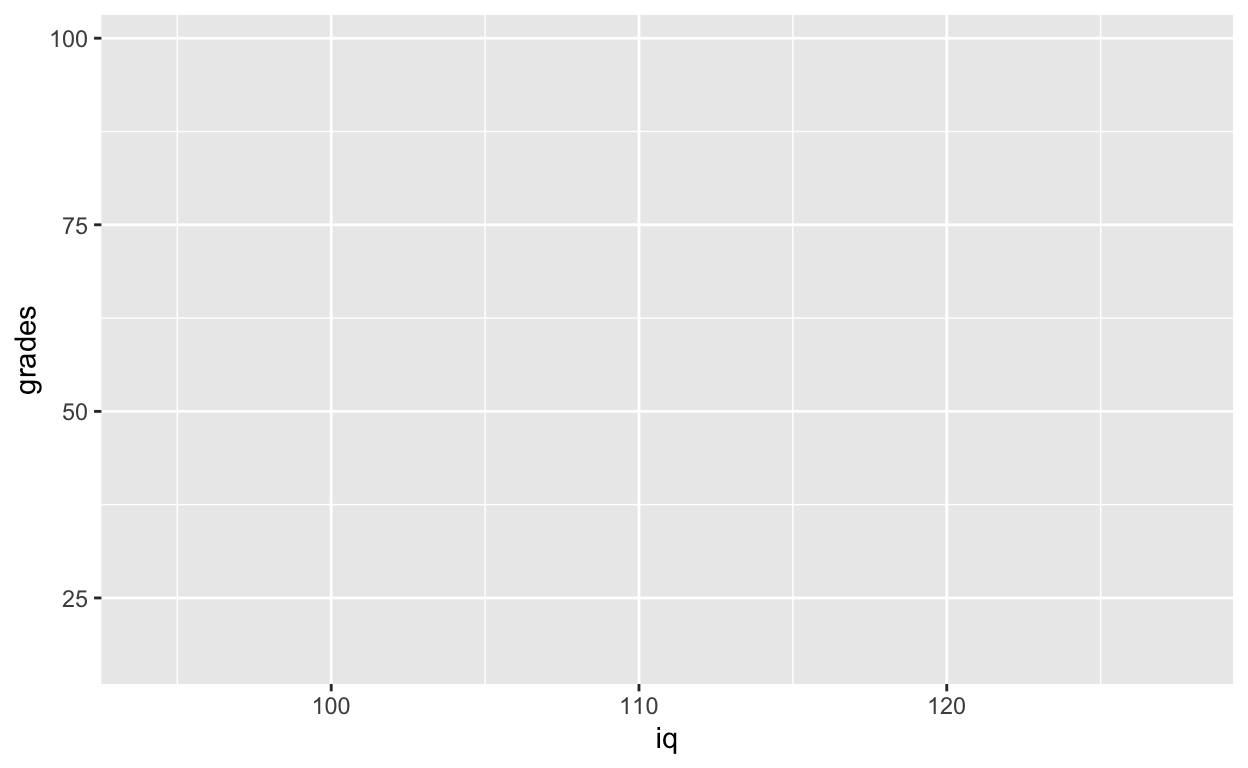### Subsequent layers: add data points and everything else

``````
ggplot(df1, aes(iq, grades)) + # also works without specifying data, x, and y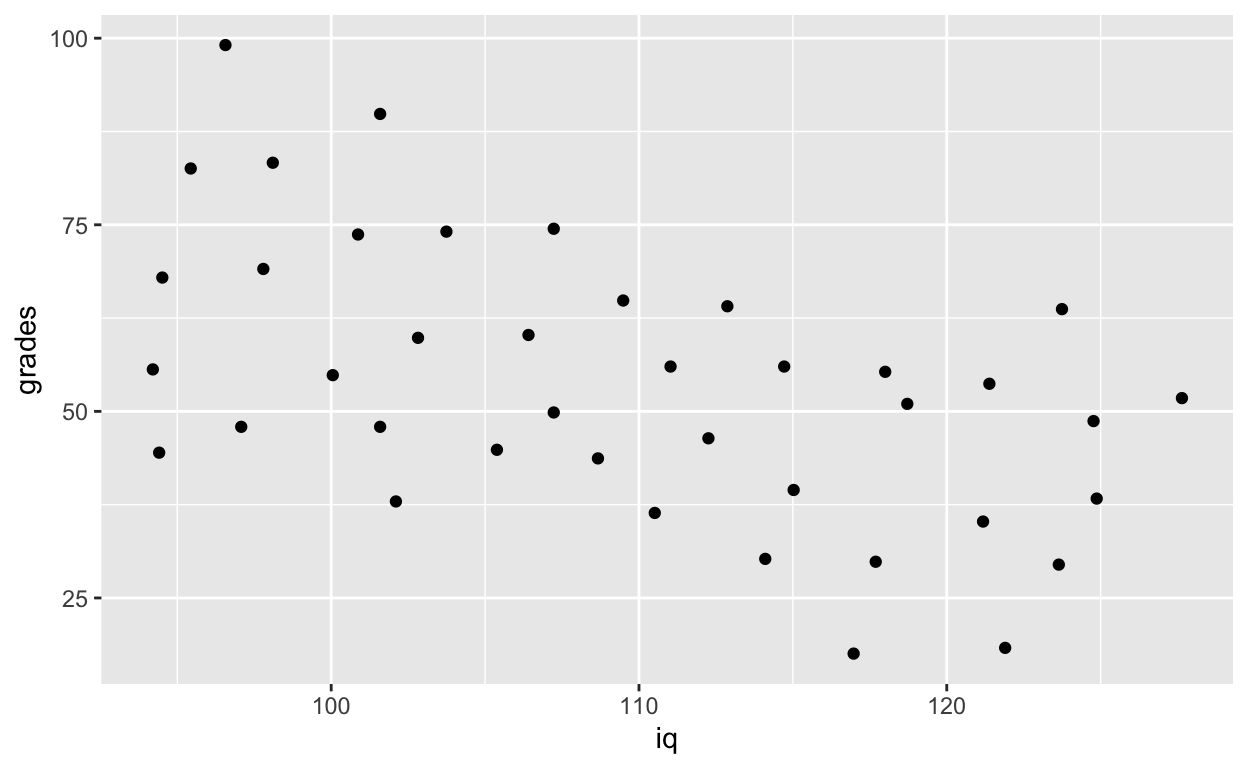Each time you want to know more about a `ggplot2` function, google ggplot2 function_name to see official documentation and examples and learn those examples! That’s usually how we plot figures. Even Hadley Wickham, the creator of `tidyverse` and many many cool things in R refers to his own online documentations all the time. There are way too many things for everyone to remember, and we usually just look them up on the internet whenever we need to use them (e.g., google ggplot2 geom point).

You’ll use `geom_point()` most frequently to add points to your plots. Check out the official documentation for `geom_point` here.

``````
geom_point(size = 8, col = 'green') + # change size and colour
labs(y = "Exam grades (0 to 100)", x = "Intelligence (IQ)") # rename axes``````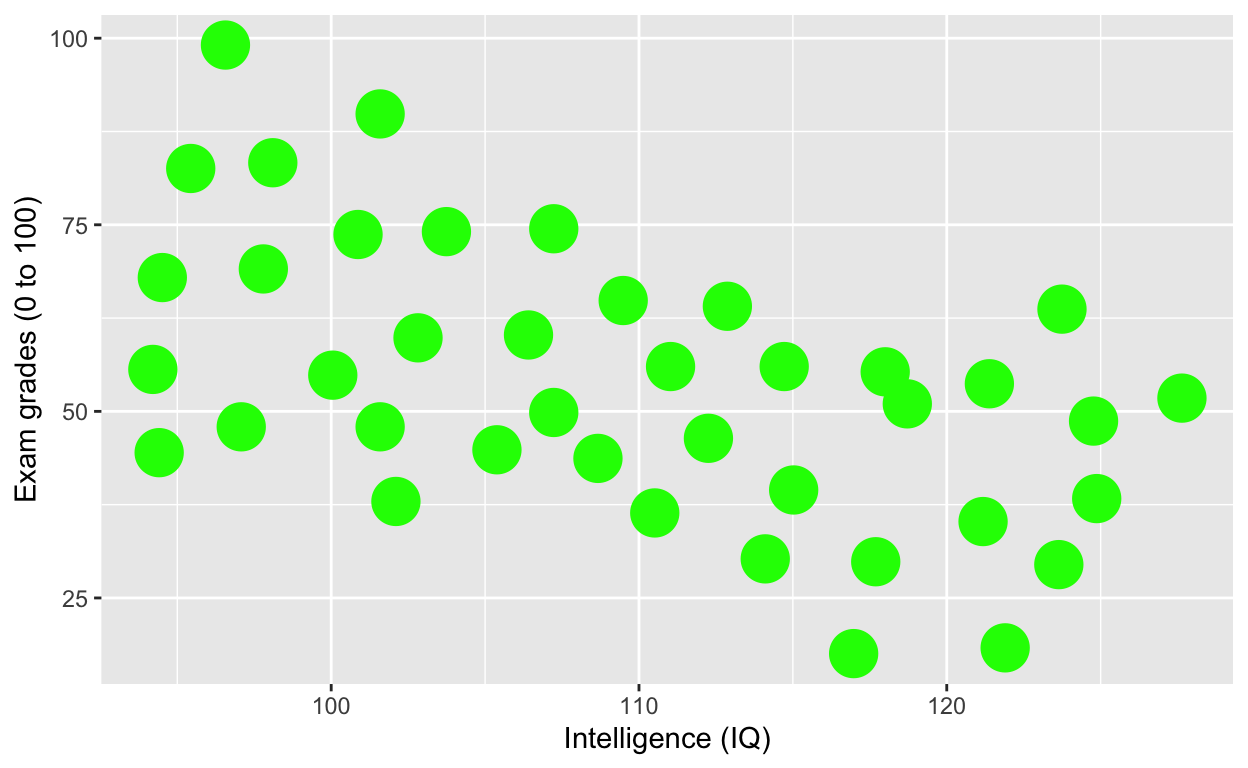``````
geom_point(size = 3, col = 'blue') + # change size and colour
labs(y = "Exam grades (0 to 100)", x = "Intelligence (IQ)") + # rename axes
scale_y_continuous(limits = c(0, 100), breaks = c(0, 20, 40, 60, 80, 100)) + # y axis limits/range (0, 100), break points
scale_x_continuous(limits = c(90, 130)) # x axis limits/range``````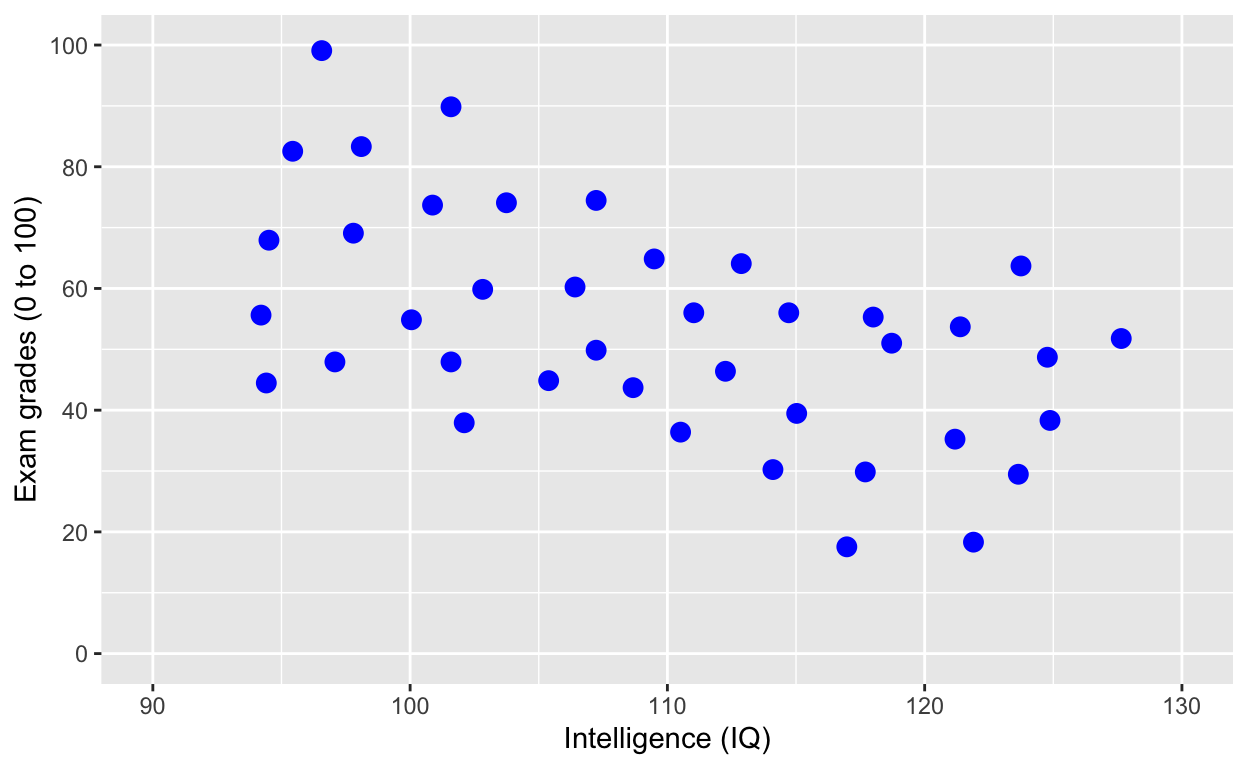## Save the plot as an object

``````
plot1 <- ggplot(df1, aes(iq, grades)) +
geom_point(size = 3, col = 'red') + # change size and colour
labs(y = "Exam grades (0 to 100)", x = "Intelligence (IQ)") + # rename axes
scale_y_continuous(limits = c(0, 100), breaks = c(0, 20, 40, 60, 80, 100)) + # y axis limits/range (0, 100), break points
scale_x_continuous(limits = c(90, 130)) # x axis limits/range
plot1 # print plot``````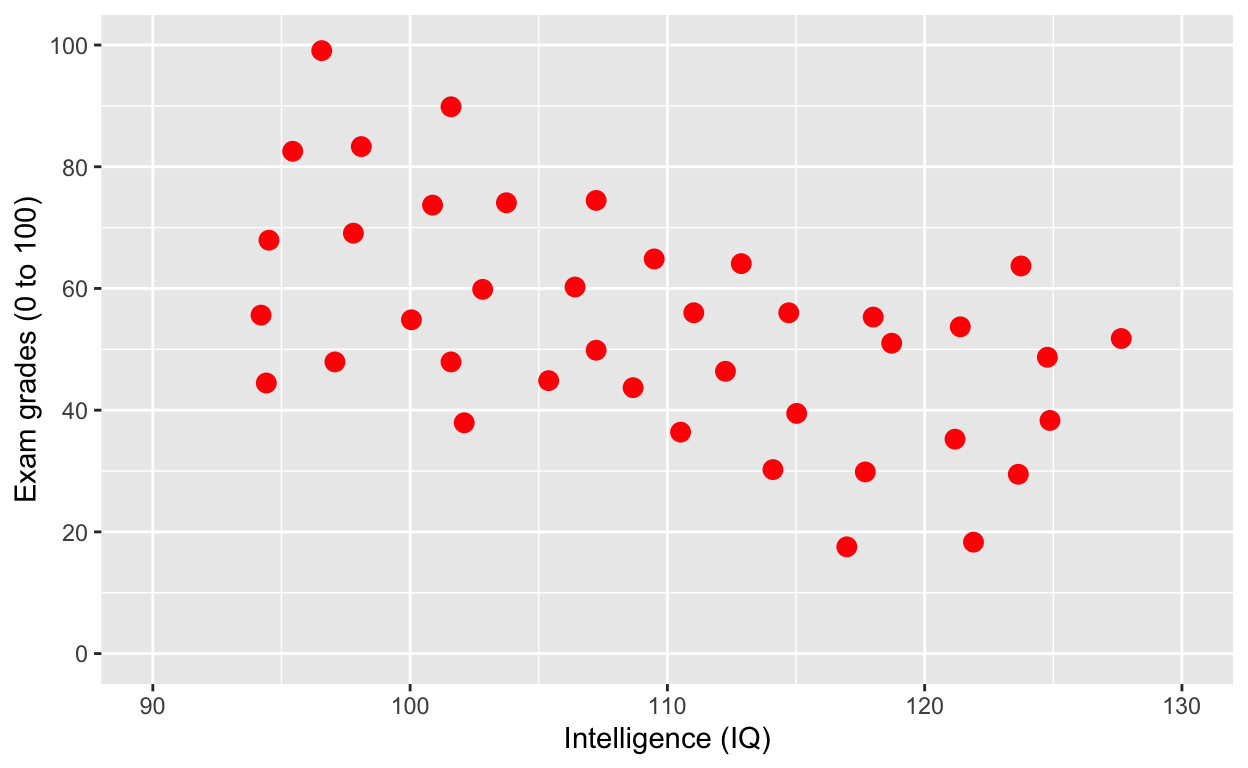## Save a plot to your directory

Save to Figures directory, assuming this directory/folder already exists. You can also change the width/height of your figure and dpi (resolution/quality) of your figure (since journals often expect around 300 dpi).

``````
ggsave(plot1, './Figures/iq_grades.png', width = 10, heigth = 10, dpi = 100)``````

## Add line of best fit

``````
plot1 +
geom_smooth() # fit line to data (defaults loess smoothing)``````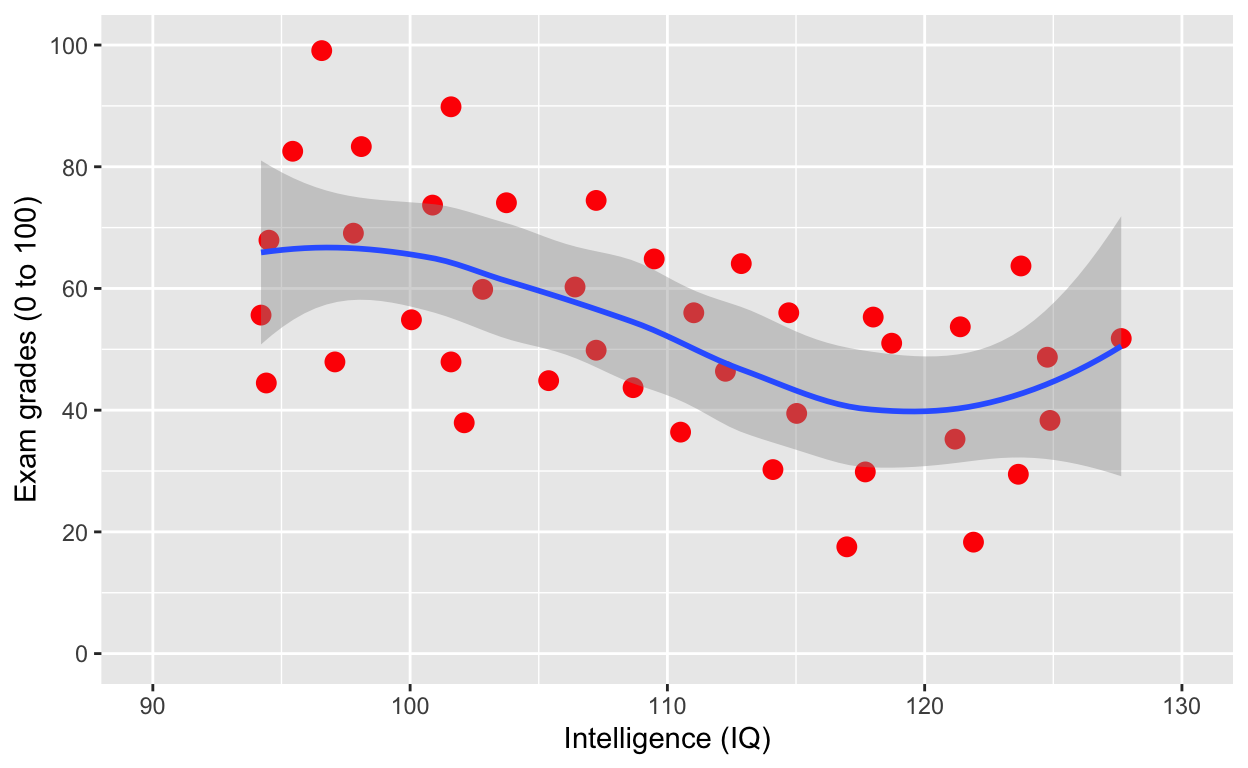Same as above

``````
geom_point(size = 3, col = 'red') + # change size and colour
labs(y = "Exam grades (0 to 100)", x = "Intelligence (IQ)") + # rename axes
scale_y_continuous(limits = c(0, 100), breaks = c(0, 20, 40, 60, 80, 100)) + # y axis limits/range (0, 100), break points
scale_x_continuous(limits = c(90, 130)) + # x axis limits/range
geom_smooth()``````Note that the smooth (i.e., the line of best fit) is on top of the dots, because of layering. Let’s add the line first, then use `geom_point()`. What do you think will happen?

``````
geom_smooth(size = 2) +
geom_point(size = 3, col = 'red') + # change size and colour
labs(y = "Exam grades (0 to 100)", x = "Intelligence (IQ)", title = 'Changed layers') + # rename axes
scale_y_continuous(limits = c(0, 100), breaks = c(0, 20, 40, 60, 80, 100)) + # y axis limits/range (0, 100), break points
scale_x_continuous(limits = c(90, 130))# x axis limits/range ``````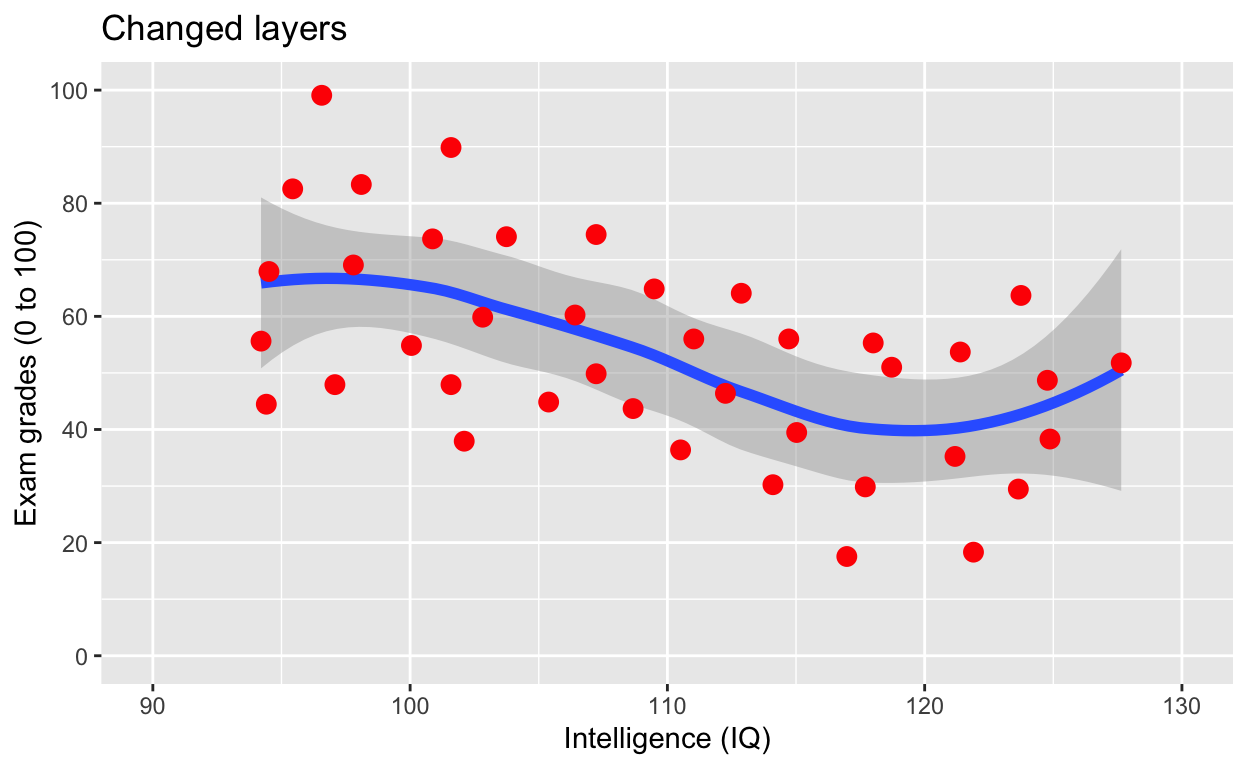Note that now the points are above the line. Also, I’ve added a title via the `labs()` line.

``````
geom_point(size = 3, col = 'red') + # change size and colour
labs(y = "Exam grades (0 to 100)", x = "Intelligence (IQ)") + # rename axes
scale_y_continuous(limits = c(0, 100), breaks = c(0, 20, 40, 60, 80, 100)) + # y axis limits/range (0, 100), break points
scale_x_continuous(limits = c(90, 130)) + # x axis limits/range
geom_smooth(method = 'lm', se = F, col = 'black') # fit linear regression line, remove standard error, black line``````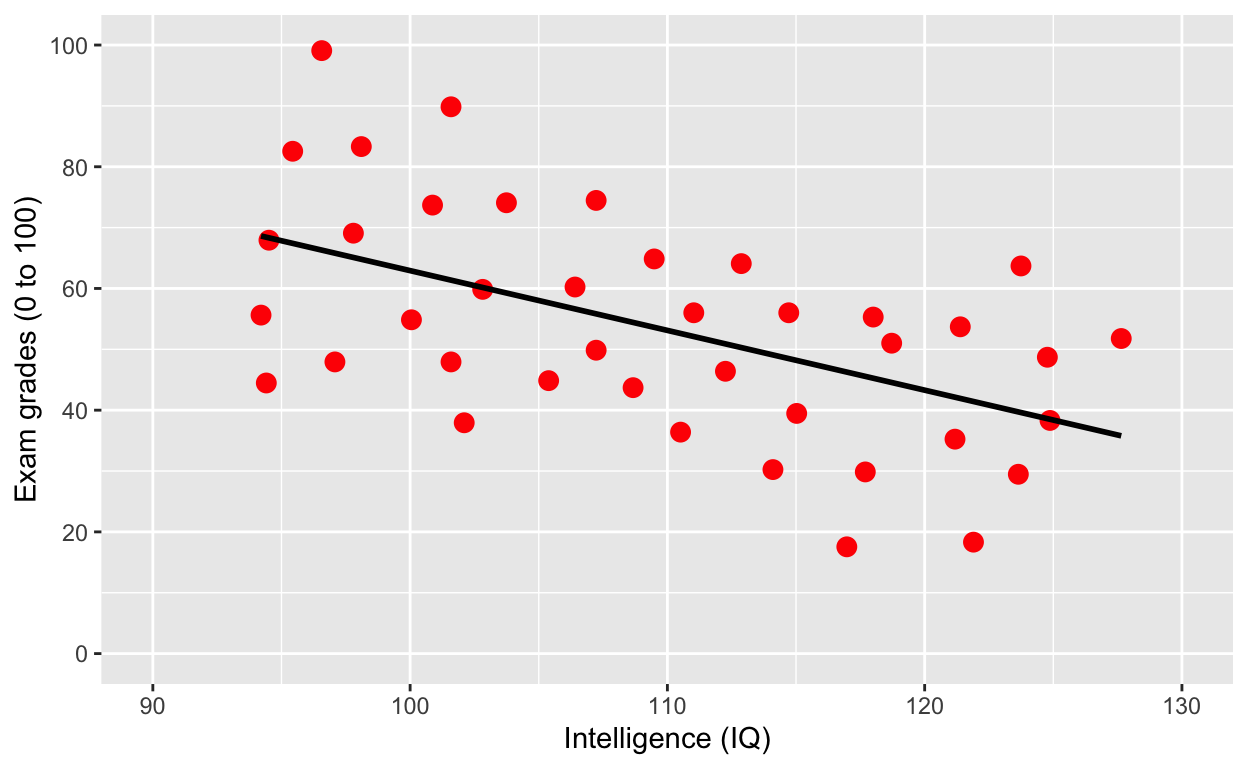Why is IQ negatively correlated with grades?

## Grouping

### Use `col` to specify grouping variable

Note what’s new in the first line/layer to add grouping.

``````
ggplot(df1, aes(iq, grades, col = class)) +
geom_point(size = 3) + # change size and colour
labs(y = "Exam grades (0 to 100)", x = "Intelligence (IQ)") + # rename axes
scale_y_continuous(limits = c(0, 100), breaks = c(0, 20, 40, 60, 80, 100)) + # y axis limits/range (0, 100), break points
scale_x_continuous(limits = c(90, 130)) + # x axis limits/range
geom_smooth(method = 'lm', se = F) # fit linear regression line ``````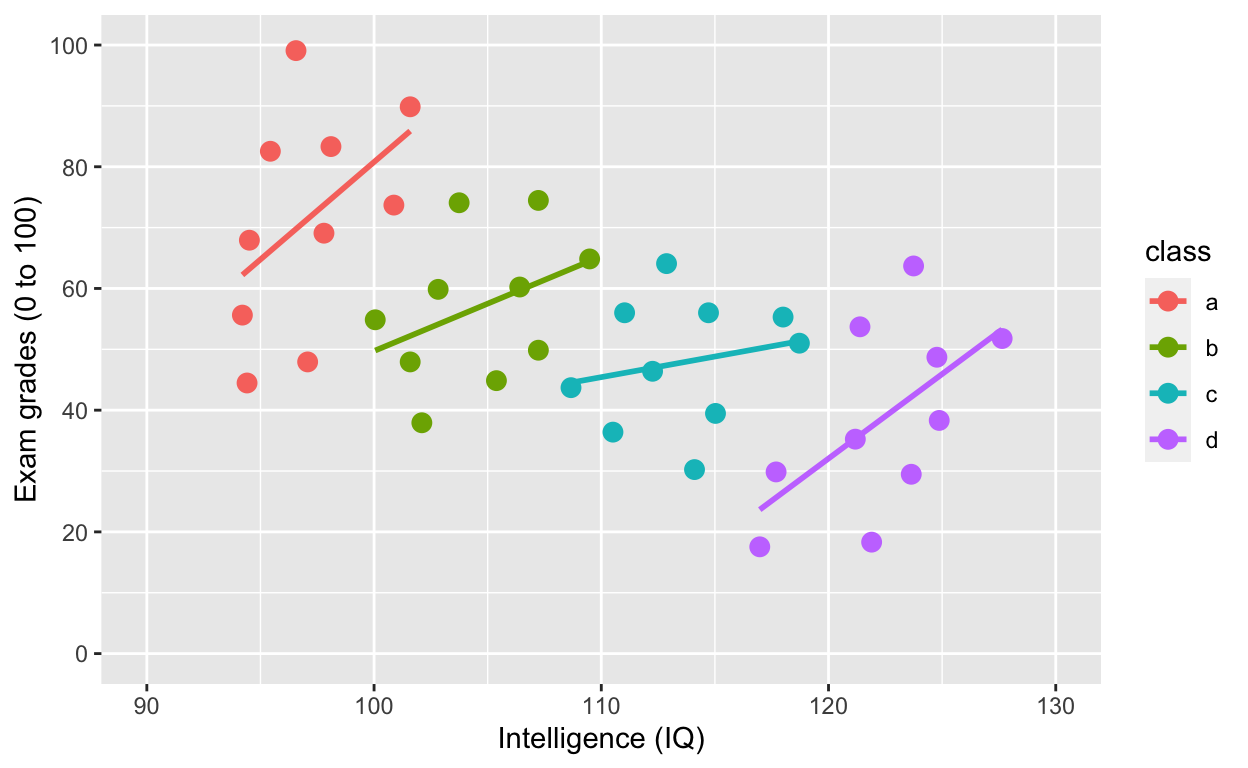`ggplot(df1, aes(iq, grades, col = class))` specifies the data to plot `df1`, x-axis `iq`, y-axis `grades`, and to give different colours to different groups `col = class`, where `class` refers to the grouping variable in the dataset.

What is the relationship between IQ and grades within each class now? What happened?!?

### Use `shape` to specify grouping variable

``````
ggplot(df1, aes(iq, grades, shape = class)) +
geom_point(size = 3) + # change size and colour
labs(y = "Exam grades (0 to 100)", x = "Intelligence (IQ)") + # rename axes
scale_y_continuous(limits = c(0, 100), breaks = c(0, 20, 40, 60, 80, 100)) + # y axis limits/range (0, 100), break points
scale_x_continuous(limits = c(90, 130)) + # x axis limits/range
geom_smooth(method = 'lm', se = F) # fit linear regression line ``````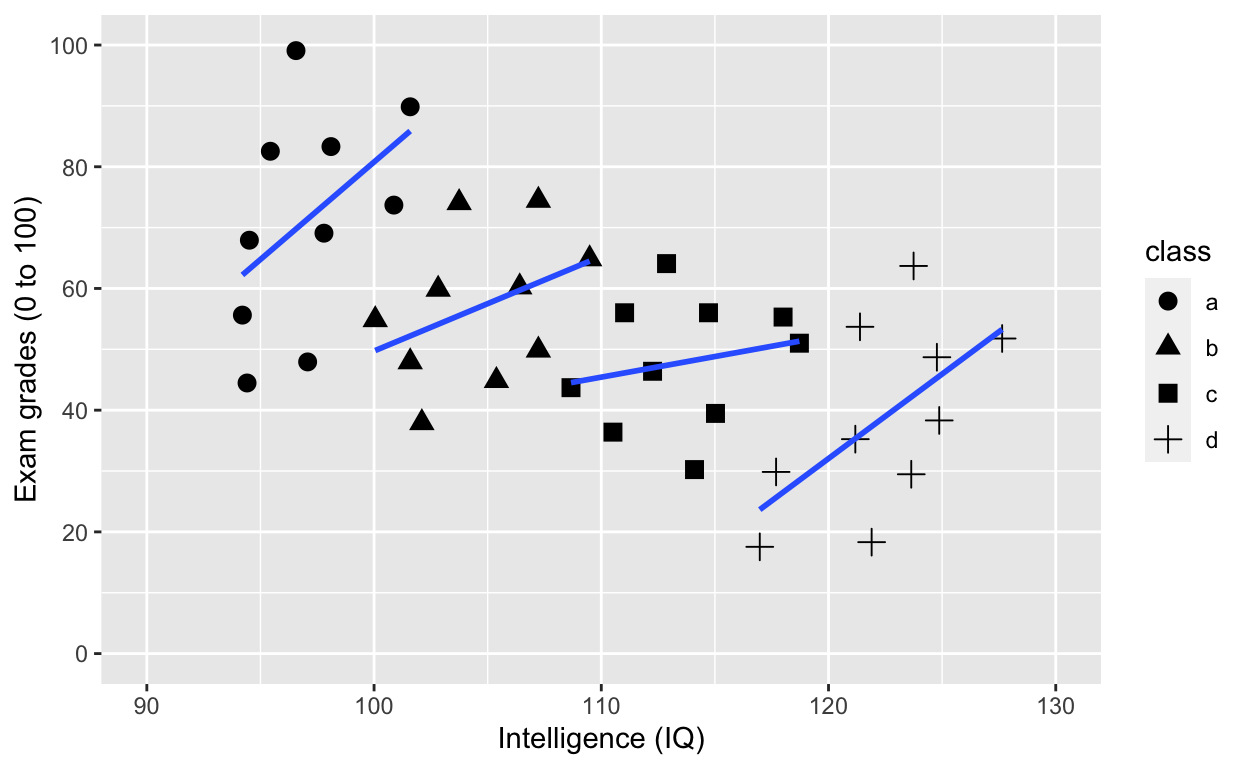### Adding an overall line of best fit while ignoring class

``````
ggplot(df1, aes(iq, grades, col = class)) +
geom_point(size = 3) + # change size and colour
labs(y = "Exam grades (0 to 100)", x = "Intelligence (IQ)") + # rename axes
scale_y_continuous(limits = c(0, 100), breaks = c(0, 20, 40, 60, 80, 100)) + # y axis limits/range (0, 100), break points
scale_x_continuous(limits = c(90, 130)) + # x axis limits/range
geom_smooth(method = 'lm', se = F, aes(group = 1)) # fit linear regression line ``````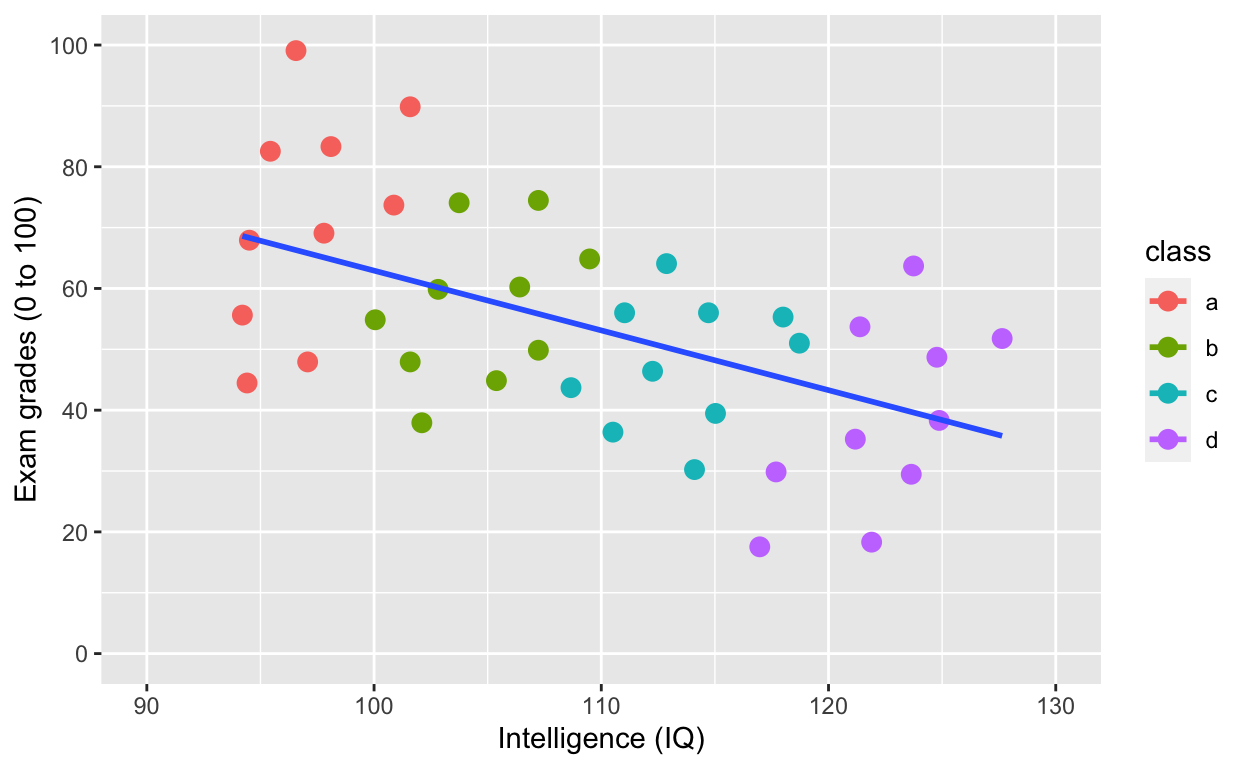### Adding an overall line of best fit AND separate lines for each group

``````
plot2 <- ggplot(df1, aes(iq, grades, col = class)) +
geom_point(size = 3) + # change size and colour
labs(y = "Exam grades (0 to 100)", x = "Intelligence (IQ)") + # rename axes
scale_y_continuous(limits = c(0, 100), breaks = c(0, 20, 40, 60, 80, 100)) + # y axis limits/range (0, 100), break points
scale_x_continuous(limits = c(90, 130)) + # x axis limits/range
geom_smooth(method = 'lm', se = F) + # fit linear regression line
geom_smooth(method = 'lm', se = F, aes(group = 1))
plot2``````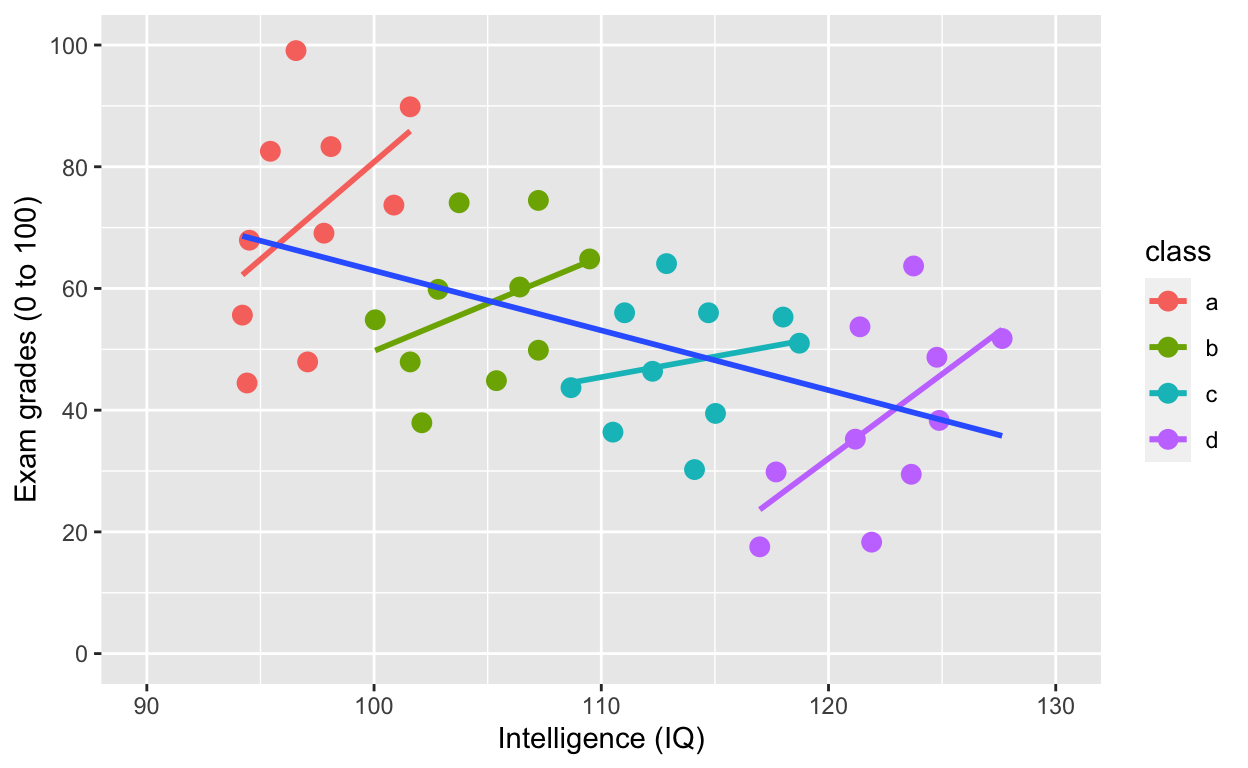Simpson’s paradox: Negative overall relationship, but positive relationship within each class.

## Plotting histograms, boxplots, and violinplots

Histogram

``````
ggplot(df1, aes(iq)) +
geom_histogram()``````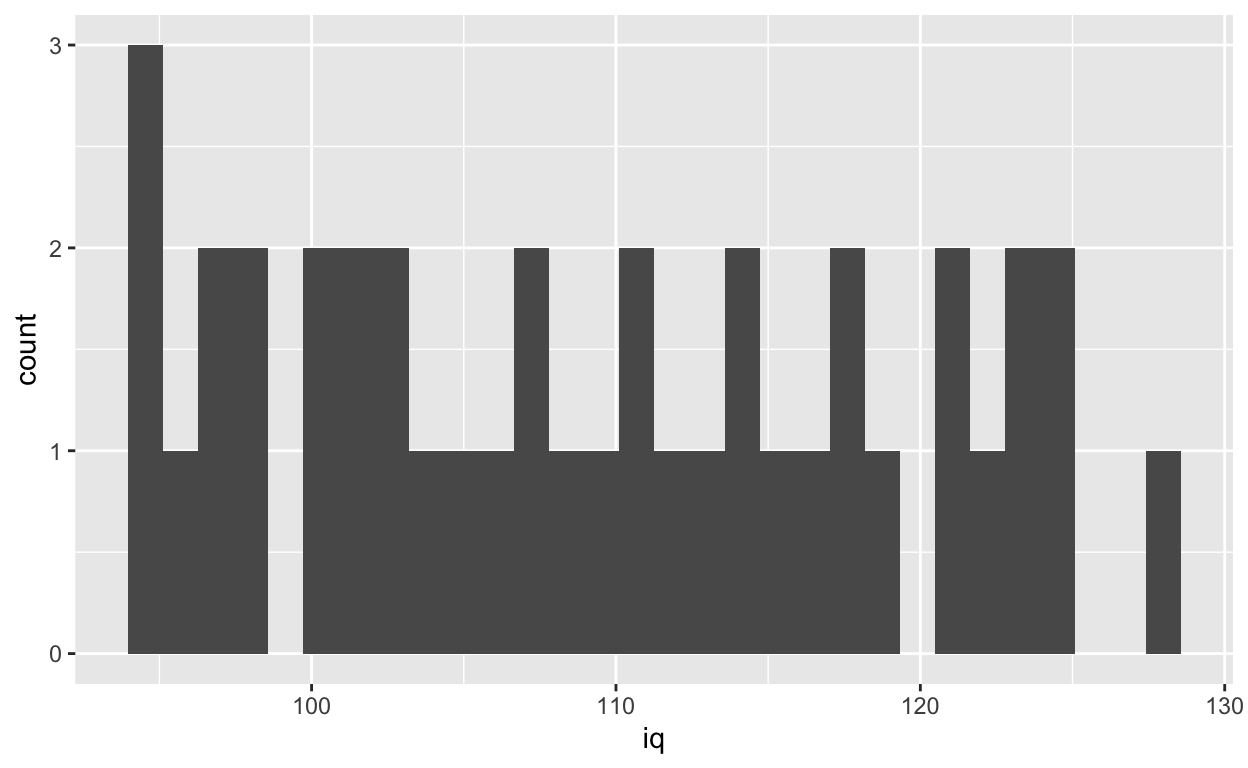Specifying binwidth

``````
ggplot(df1, aes(iq)) +
geom_histogram(binwidth = 5)``````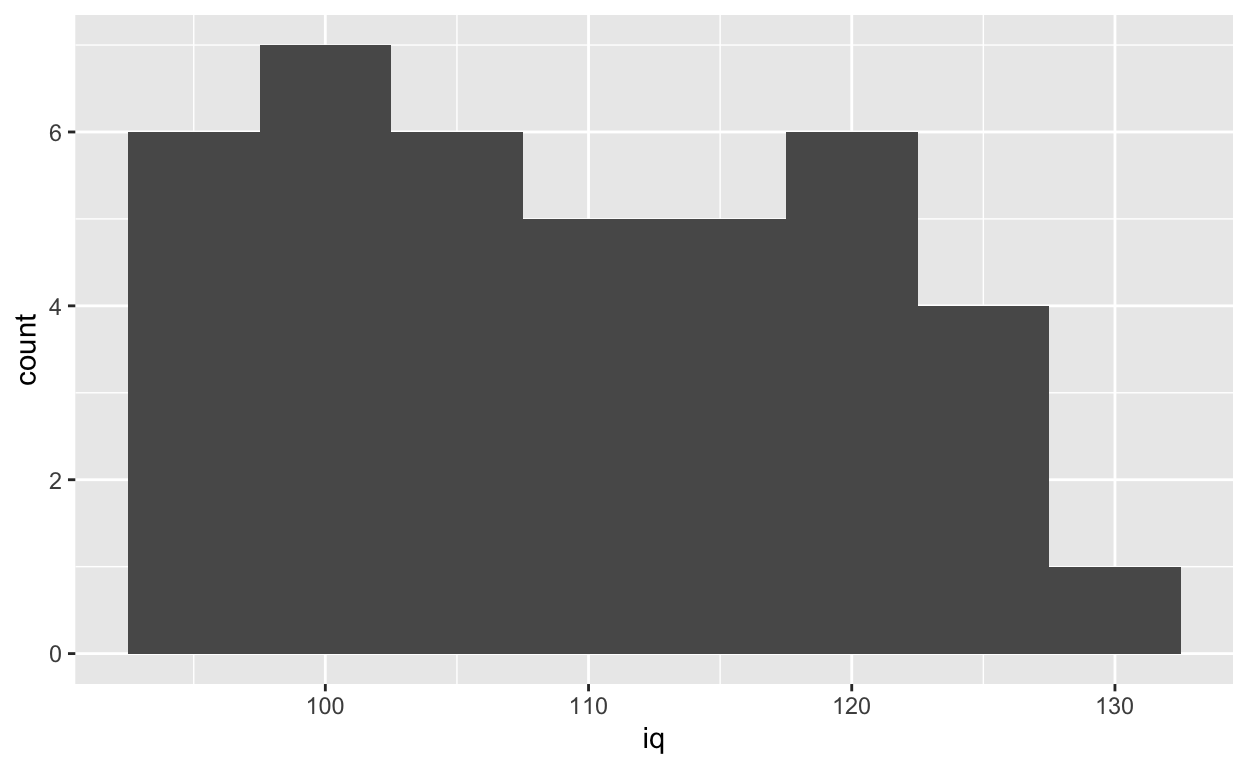Density plot

``````
ggplot(df1, aes(iq)) +
geom_density()``````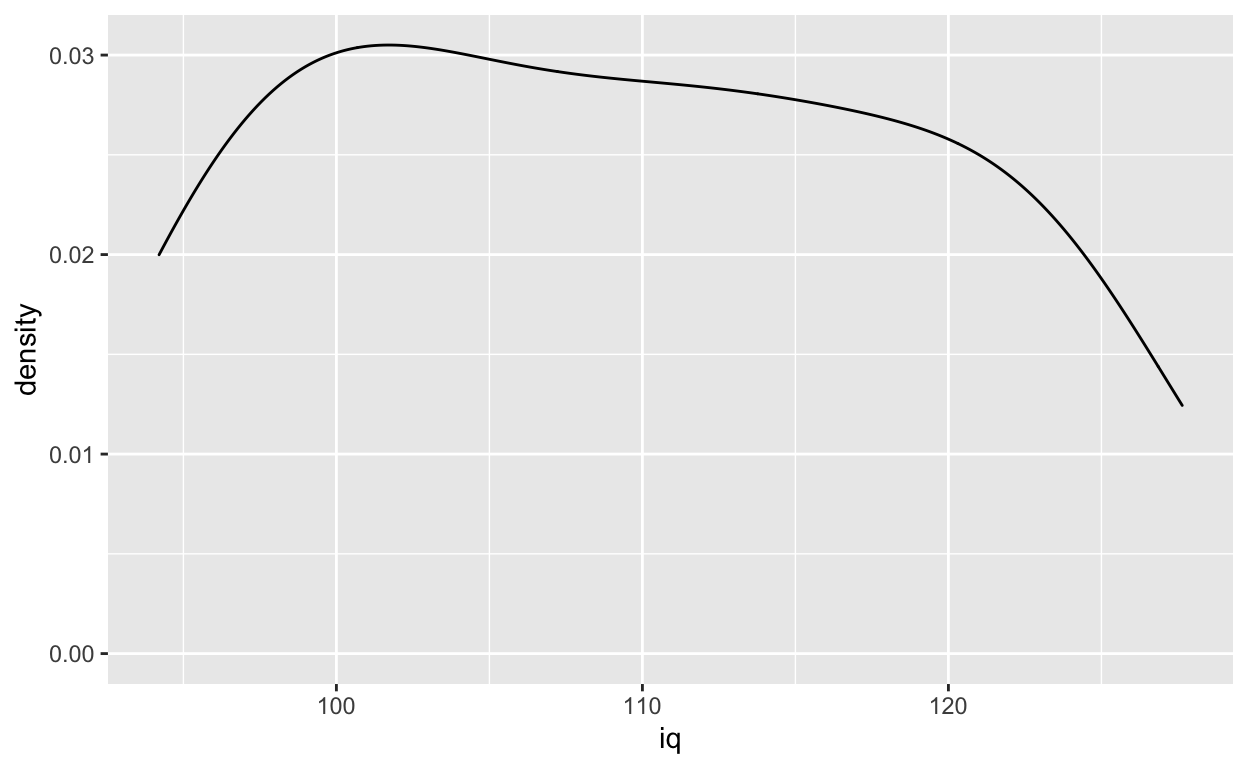Boxplot for each class

``````
geom_boxplot()``````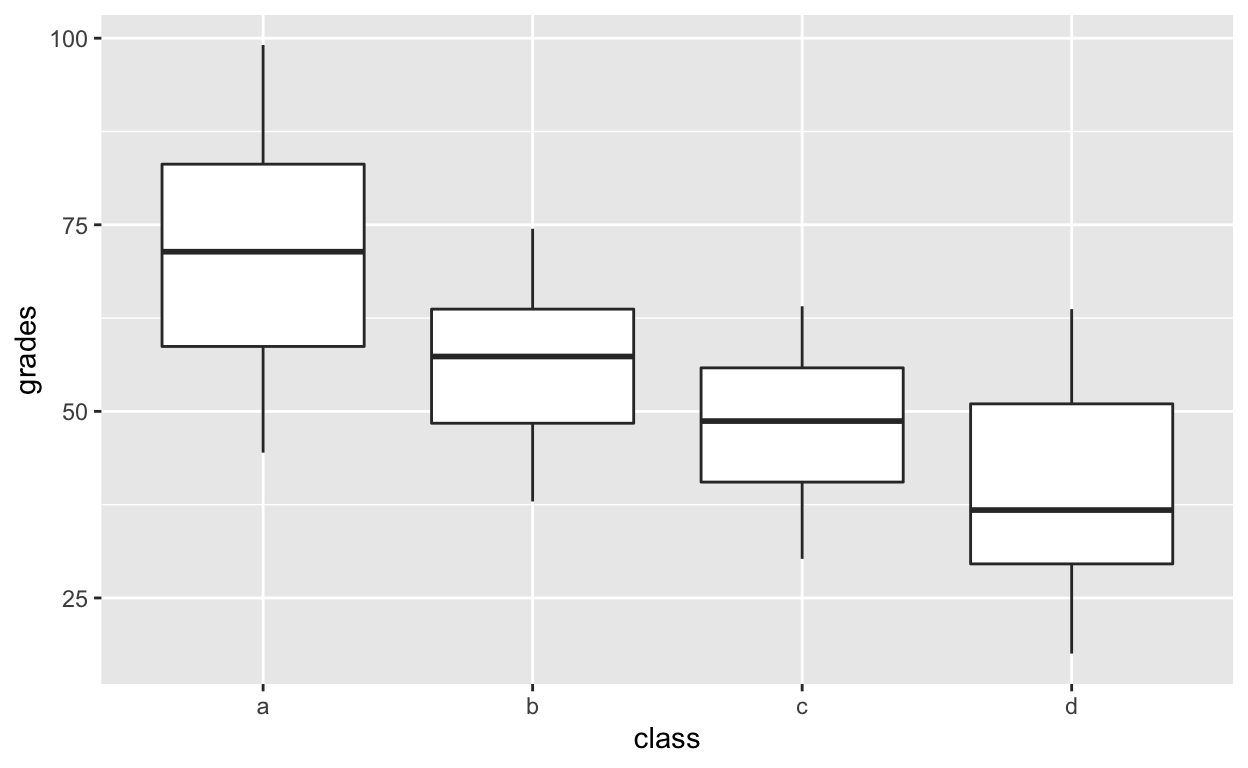Violinplot for each class

``````
geom_violin()``````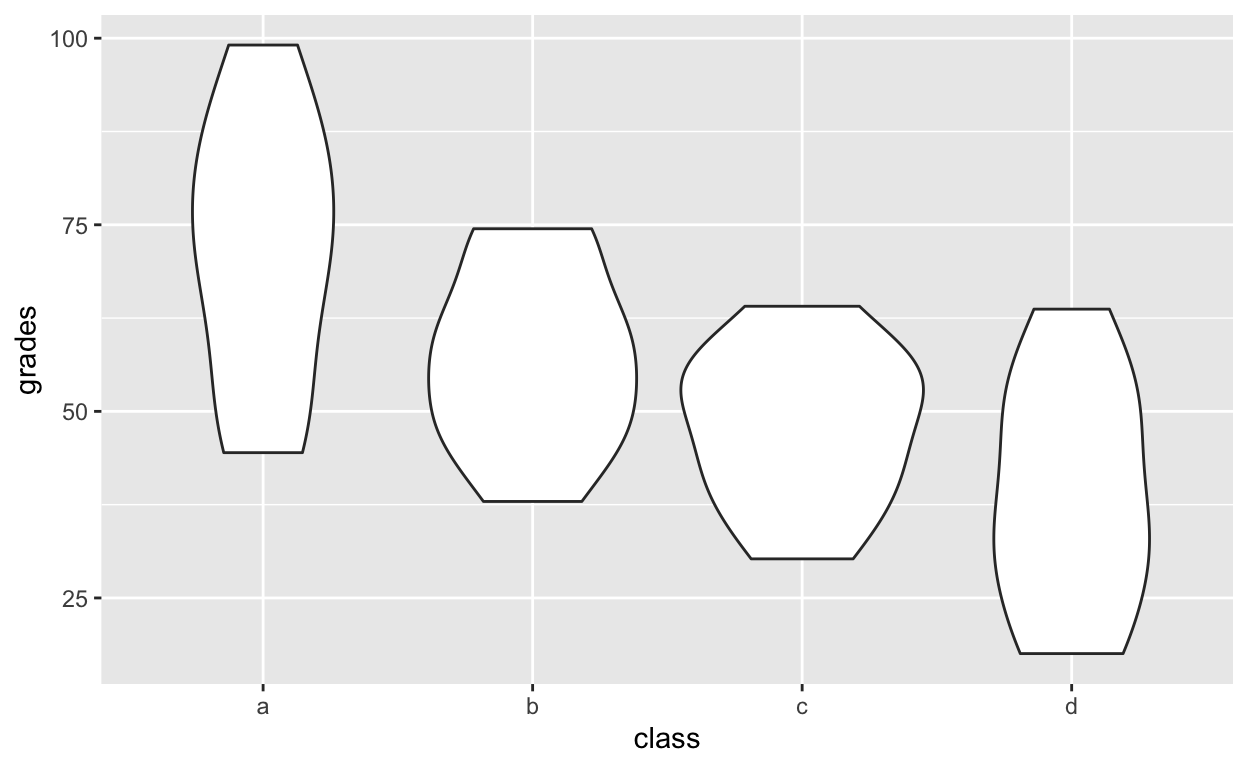Layering and colouring plots

``````
ggplot(df1, aes(class, grades, col = class)) +
geom_violin() +
geom_boxplot() +
geom_point()``````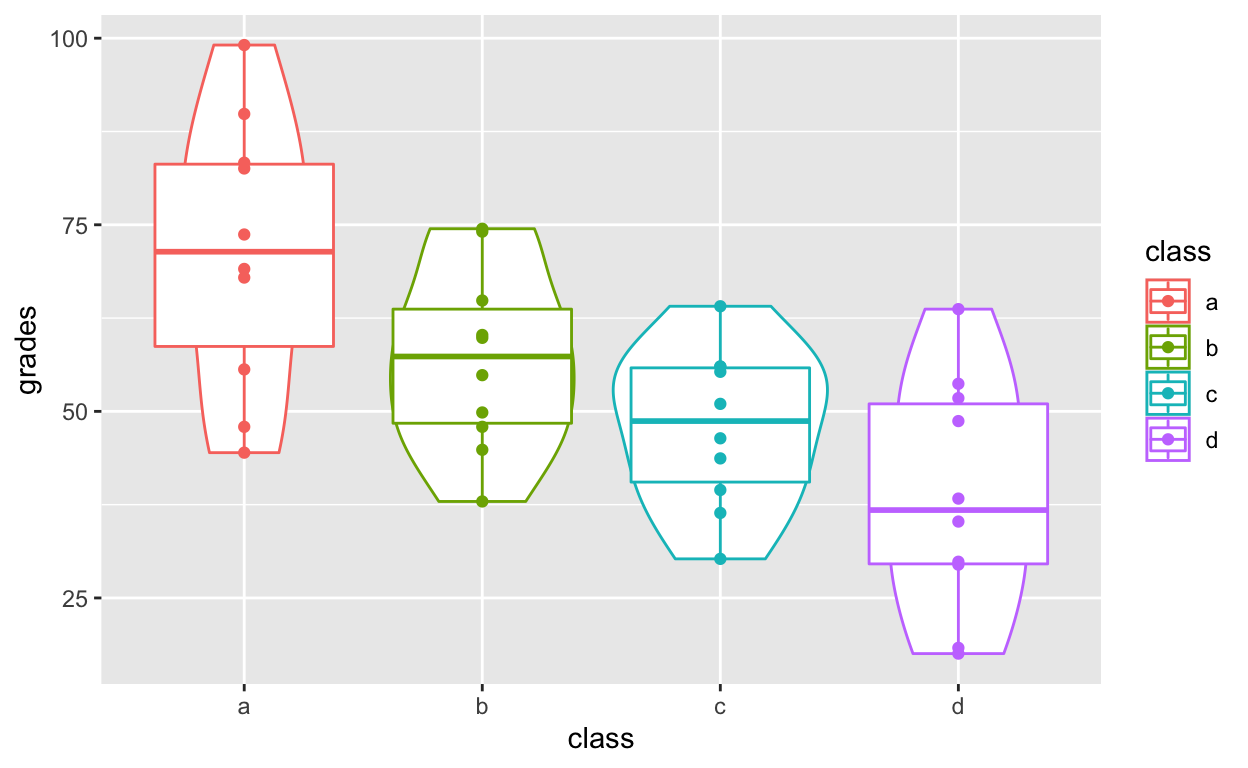## Distribution of points with `geom_quasirandom()`

An alternative that I prefer more than both boxplots and violin plots: `geom_quasirandom()` from the `ggbeeswarm` package. See here for more information.

`geom_quasirandom()` extends `geom_point()` by showing the distribution information at the same time. It basically combines all the good things in `geom_boxplot`, `geom_violin`, `geom_point` and `geom_histogram`.

``````
ggplot(df1, aes(class, grades, col = class)) +
geom_quasirandom()``````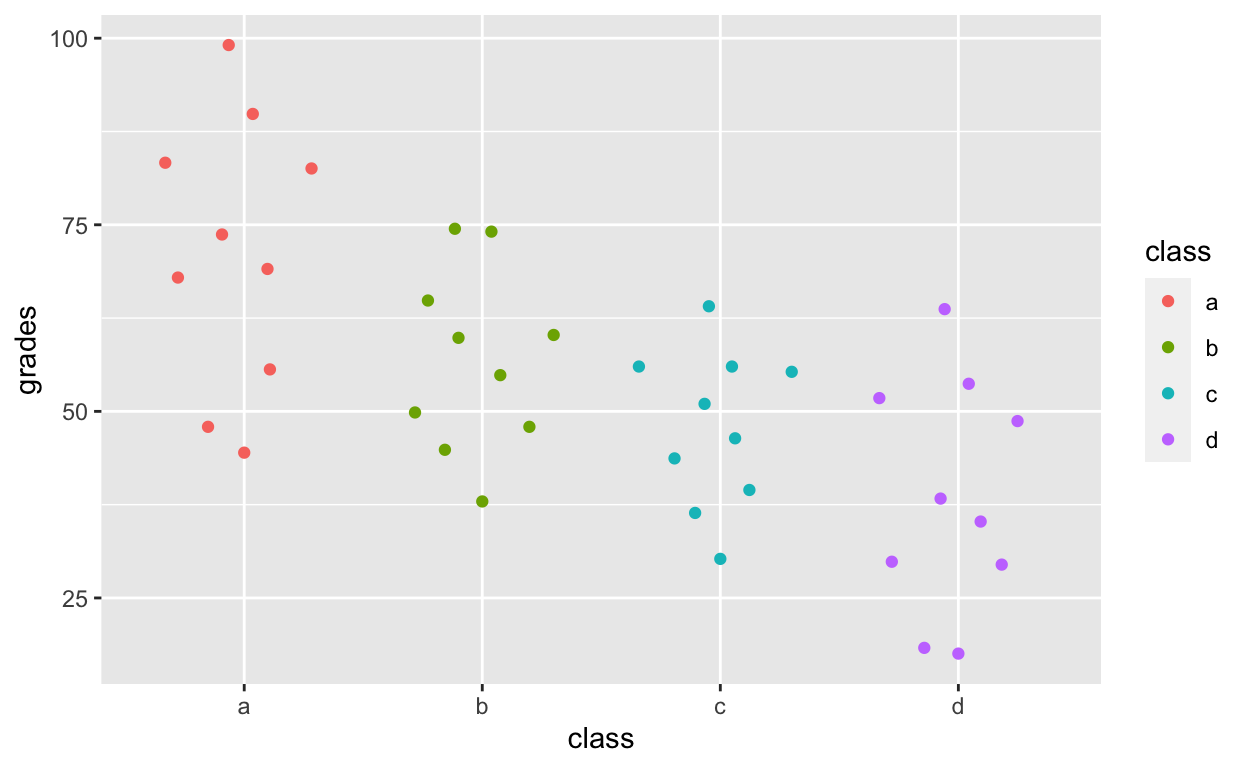``````
df1\$overallClass <- "one_class" # create variable that assigns everyone to one class
# df1[, overallClass := "one_class"] # data.table syntax for the line above``````

`geom_quasirandom` shows distribution information!

``````
geom_quasirandom()``````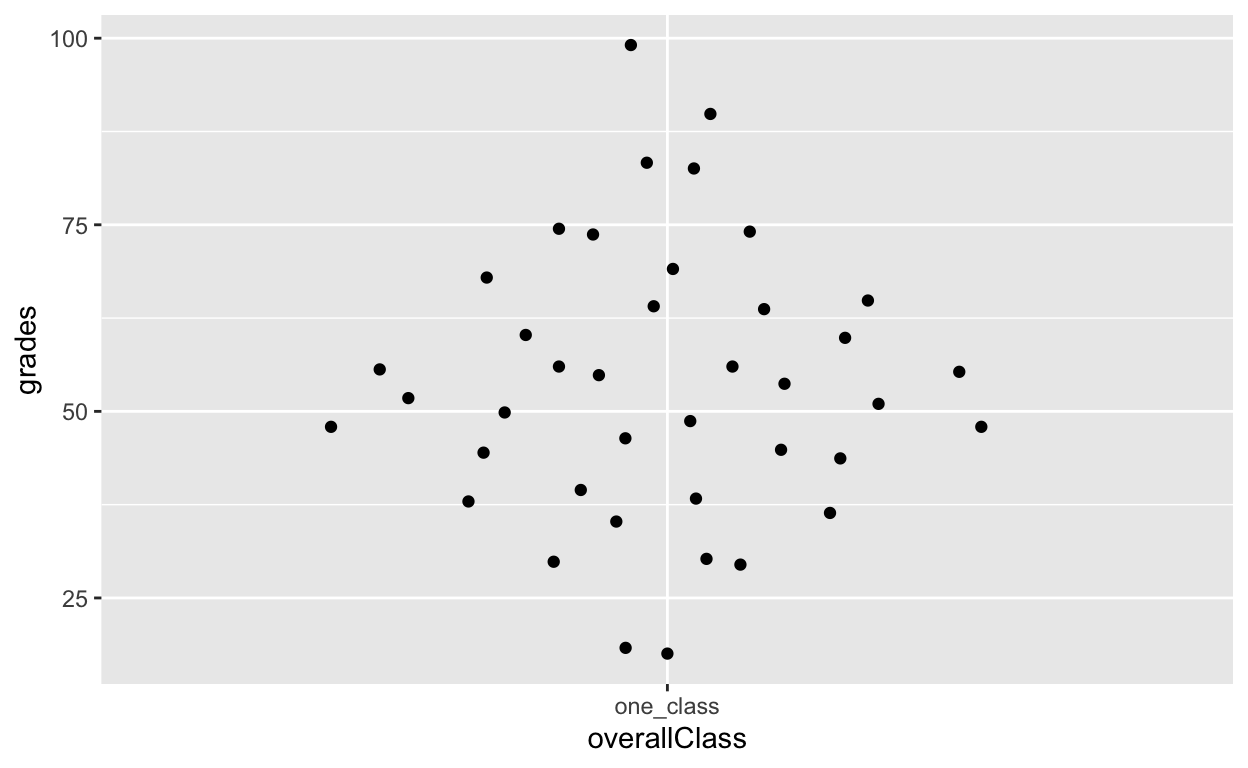``````
ggplot(df1, aes(overallClass, iq)) + # y: iq
geom_quasirandom() +
labs(x = "") # remove x-axis label (compare with above)``````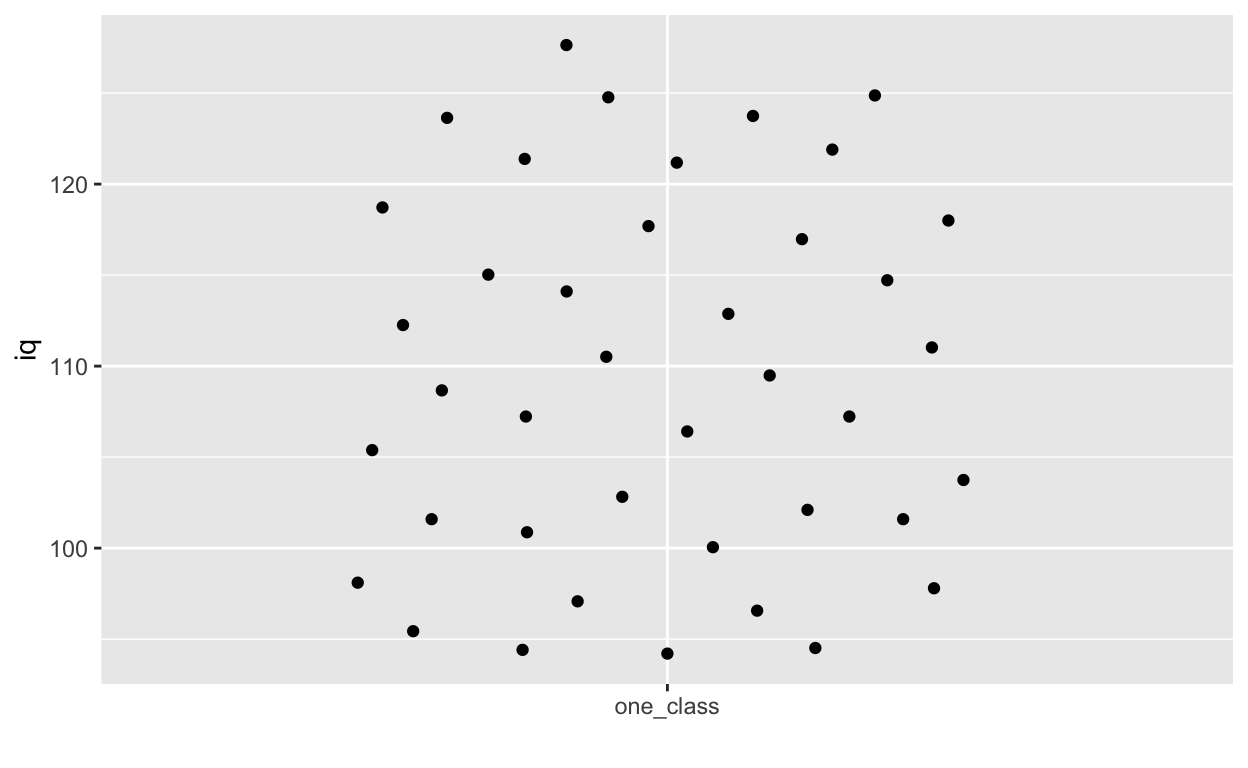## Summary statistics with ggplot2

`stat_summary()` can quickly help you compute summary statistics and plot them. If you get a warning message about Hmisc package, just install that package using `install.packages('Hmisc')` and then `library(Hmisc)`

``````
ggplot(df1, aes(class, iq)) + # y: iq
geom_quasirandom(alpha = 0.3) +
stat_summary(fun = mean, geom = 'point', size = 3) + # apply mean function (fun = mean) (median or other functions work too)
stat_summary(fun.data = mean_cl_normal, geom = 'errorbar', width = 0, size = 1) # apply mean_cl_normal function to data``````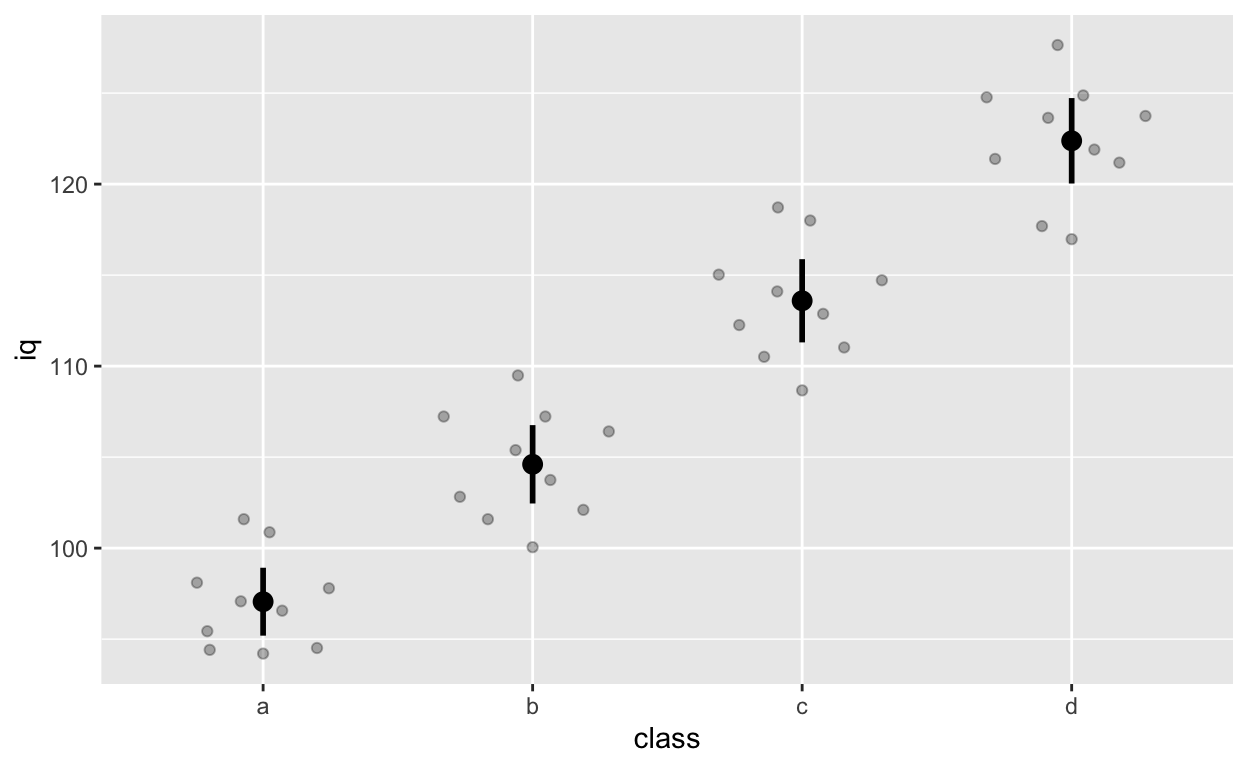## Facets for grouping: `facet_wrap()` and `facet_grid()`

Randomly assign gender to each row (see previous tutorial for detailed explanation of the code below)

``````
df1\$gender <- sample(x = c("female", "male"), size = 40, replace = T)``````

Code from before

``````
geom_point(size = 3) + # change size and colour
labs(y = "Exam grades (0 to 100)", x = "Intelligence (IQ)") + # rename axes
scale_y_continuous(limits = c(0, 100), breaks = c(0, 20, 40, 60, 80, 100)) + # y axis limits/range (0, 100), break points
scale_x_continuous(limits = c(90, 130)) + # x axis limits/range
geom_smooth(method = 'lm', se = F)``````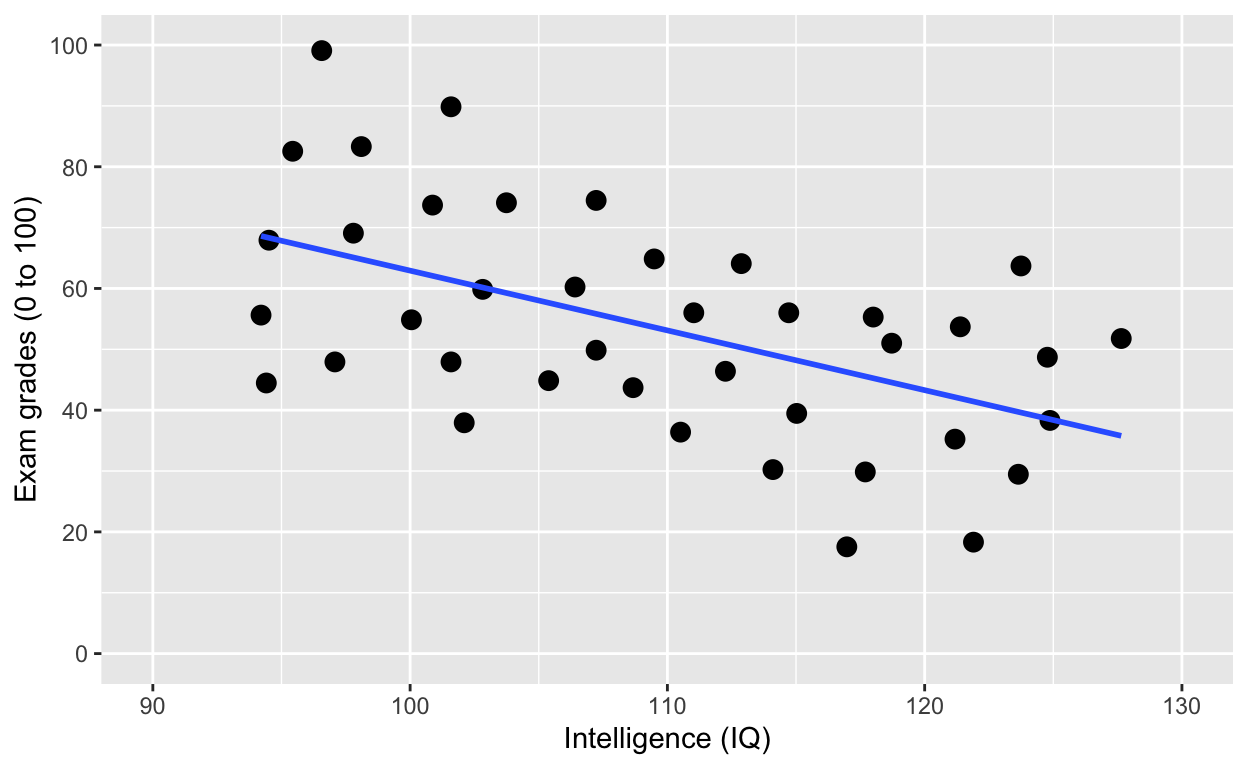Using facets instead of `col = class`. See the last line of code `facet_wrap()`.

`facet_wrap()`: one facet per class

``````
geom_point(size = 2) + # change size and colour
labs(y = "Exam grades (0 to 100)", x = "Intelligence (IQ)") + # rename axes
scale_y_continuous(limits = c(0, 100), breaks = c(0, 20, 40, 60, 80, 100)) + # y axis limits/range (0, 100), break points
scale_x_continuous(limits = c(90, 130)) + # x axis limits/range
geom_smooth(method = 'lm', se = F) +
facet_wrap(~class) # one facet per class``````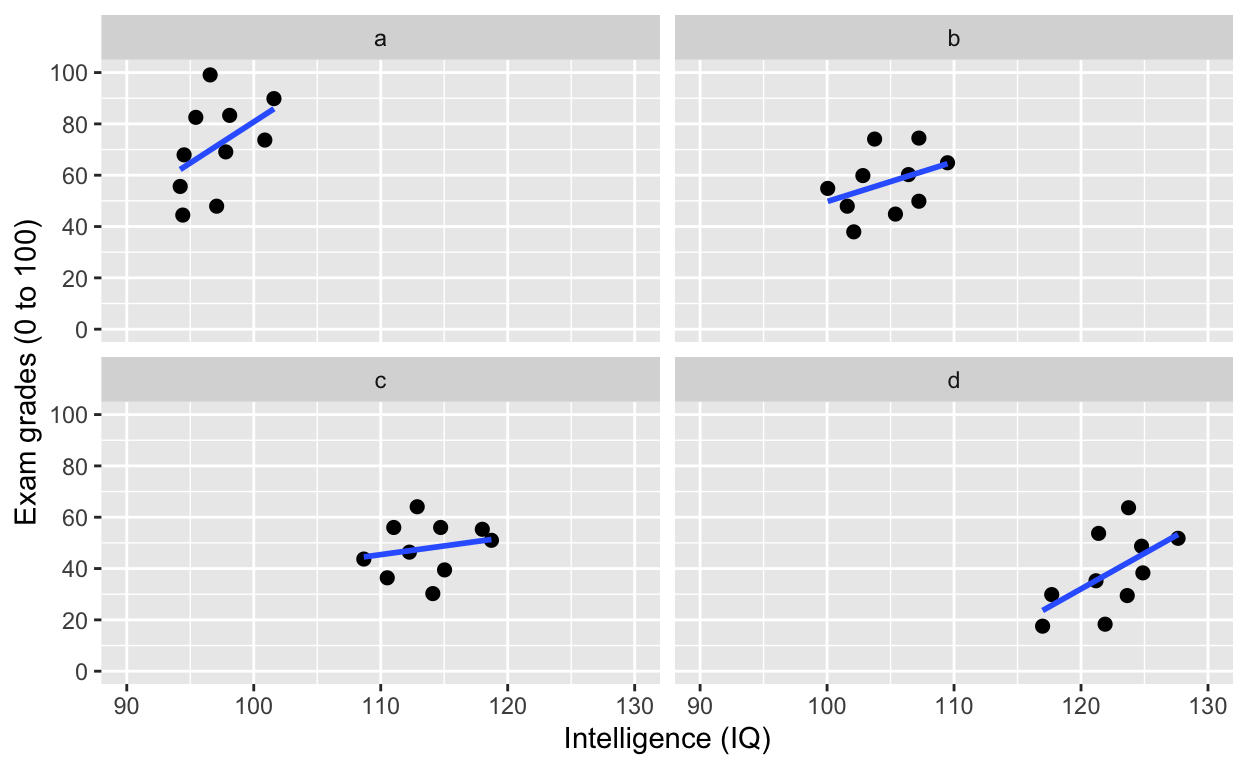`facet_wrap()`: one facet per class and gender

``````
geom_point(size = 2) + # change size and colour
labs(y = "Exam grades (0 to 100)", x = "Intelligence (IQ)") + # rename axes
scale_y_continuous(limits = c(0, 100), breaks = c(0, 20, 40, 60, 80, 100)) + # y axis limits/range (0, 100), break points
scale_x_continuous(limits = c(90, 130)) + # x axis limits/range
geom_smooth(method = 'lm', se = F) +
facet_wrap(class~gender) # one facet per class and gender``````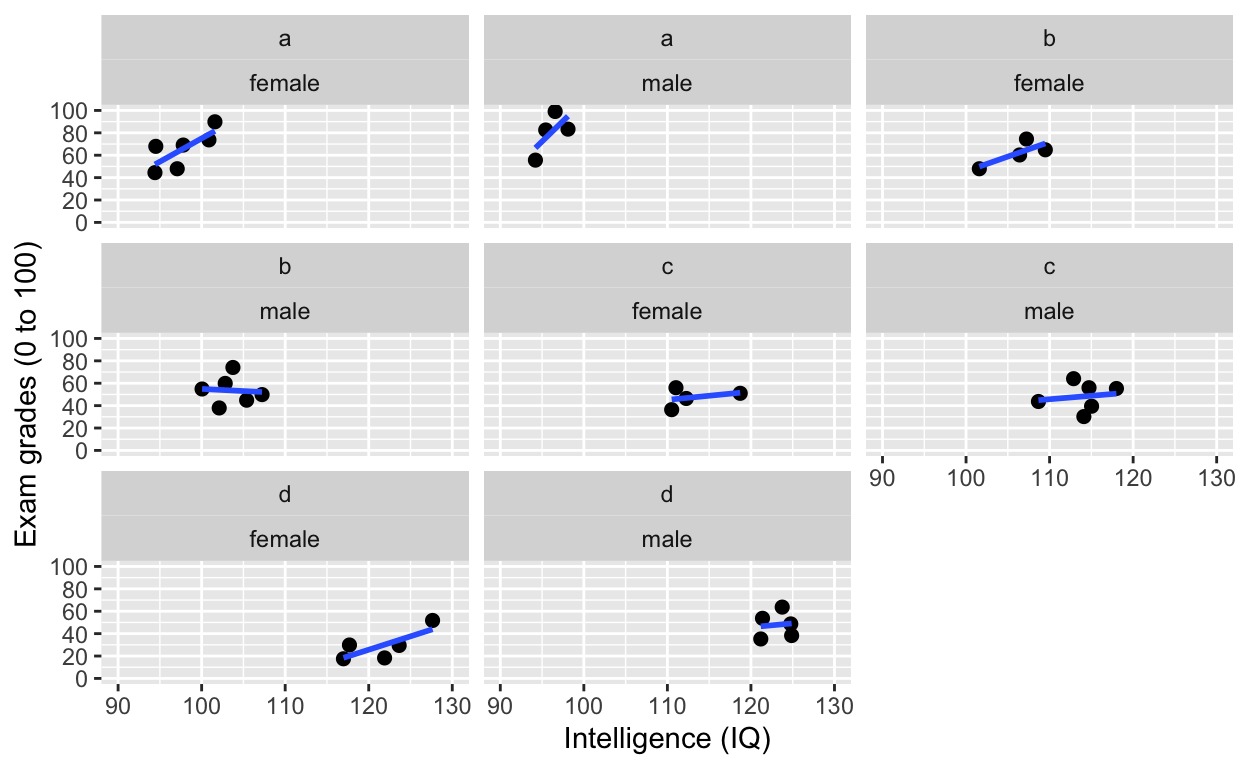`facet_grid()`: one facet per gender

``````
geom_point(size = 2) + # change size and colour
labs(y = "Exam grades (0 to 100)", x = "Intelligence (IQ)") + # rename axes
scale_y_continuous(limits = c(0, 100), breaks = c(0, 20, 40, 60, 80, 100)) + # y axis limits/range (0, 100), break points
scale_x_continuous(limits = c(90, 130)) + # x axis limits/range
geom_smooth(method = 'lm', se = F) +
facet_grid(.~gender) # one facet per gender``````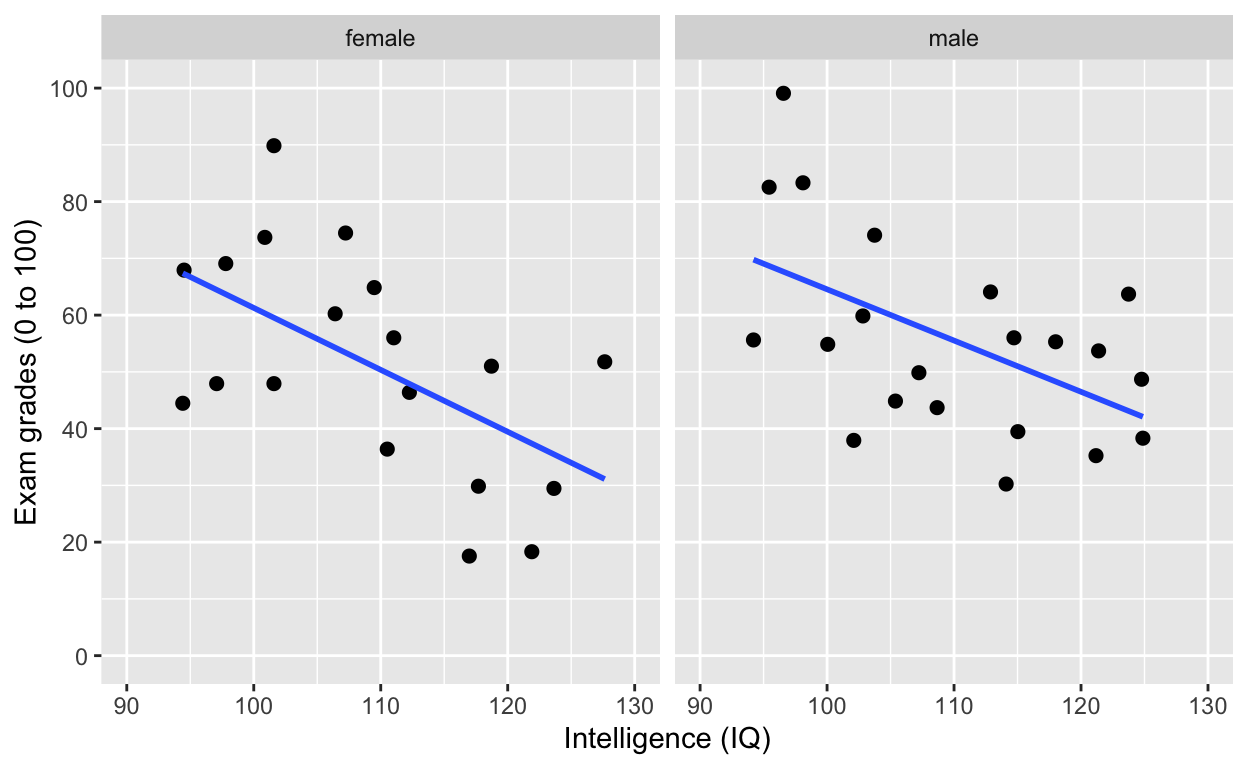`facet_grid()`: one facet per gender

``````
geom_point(size = 2) + # change size and colour
labs(y = "Exam grades (0 to 100)", x = "Intelligence (IQ)") + # rename axes
scale_y_continuous(limits = c(0, 100), breaks = c(0, 20, 40, 60, 80, 100)) + # y axis limits/range (0, 100), break points
scale_x_continuous(limits = c(90, 130)) + # x axis limits/range
geom_smooth(method = 'lm', se = F) +
facet_grid(gender~.) # one facet per gender``````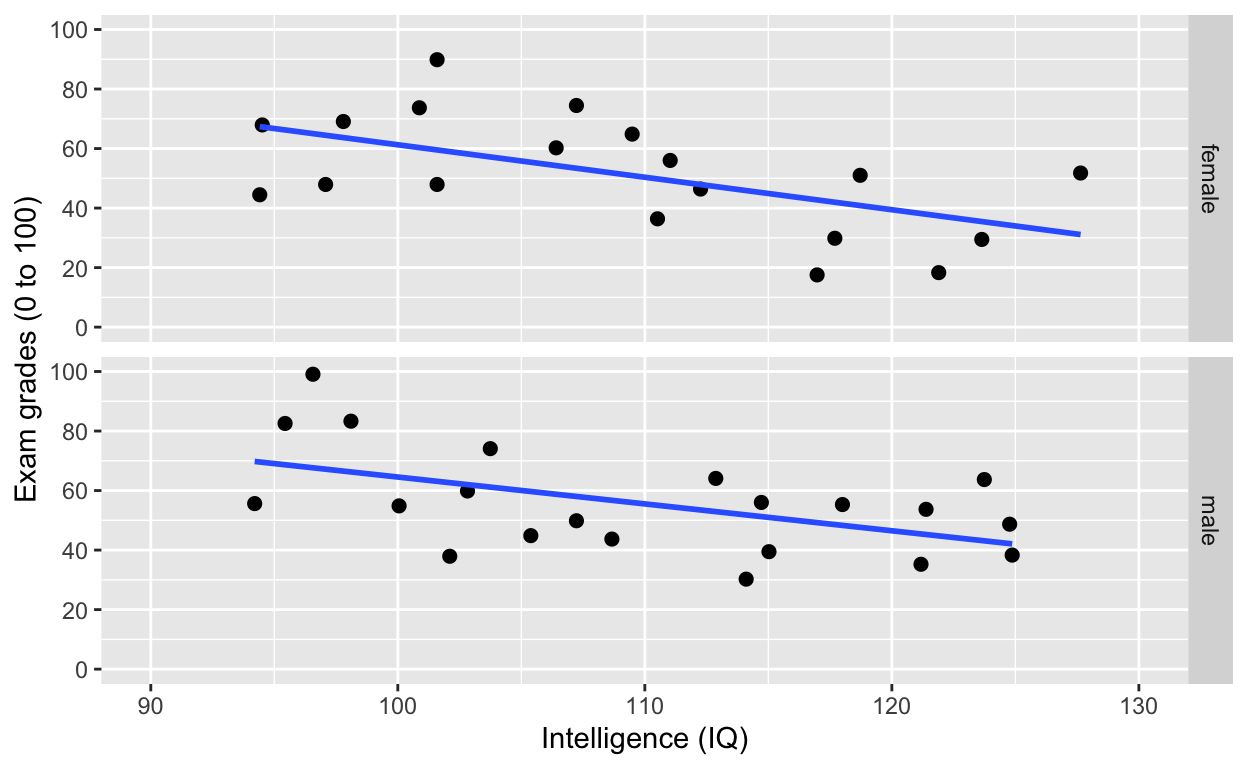`facet_grid()`: one facet per class and gender

``````
geom_point(size = 2) + # change size and colour
labs(y = "Exam grades (0 to 100)", x = "Intelligence (IQ)") + # rename axes
scale_y_continuous(limits = c(0, 100), breaks = c(0, 20, 40, 60, 80, 100)) + # y axis limits/range (0, 100), break points
scale_x_continuous(limits = c(90, 130)) + # x axis limits/range
geom_smooth(method = 'lm', se = F) +
facet_grid(gender~class) # one facet per gender``````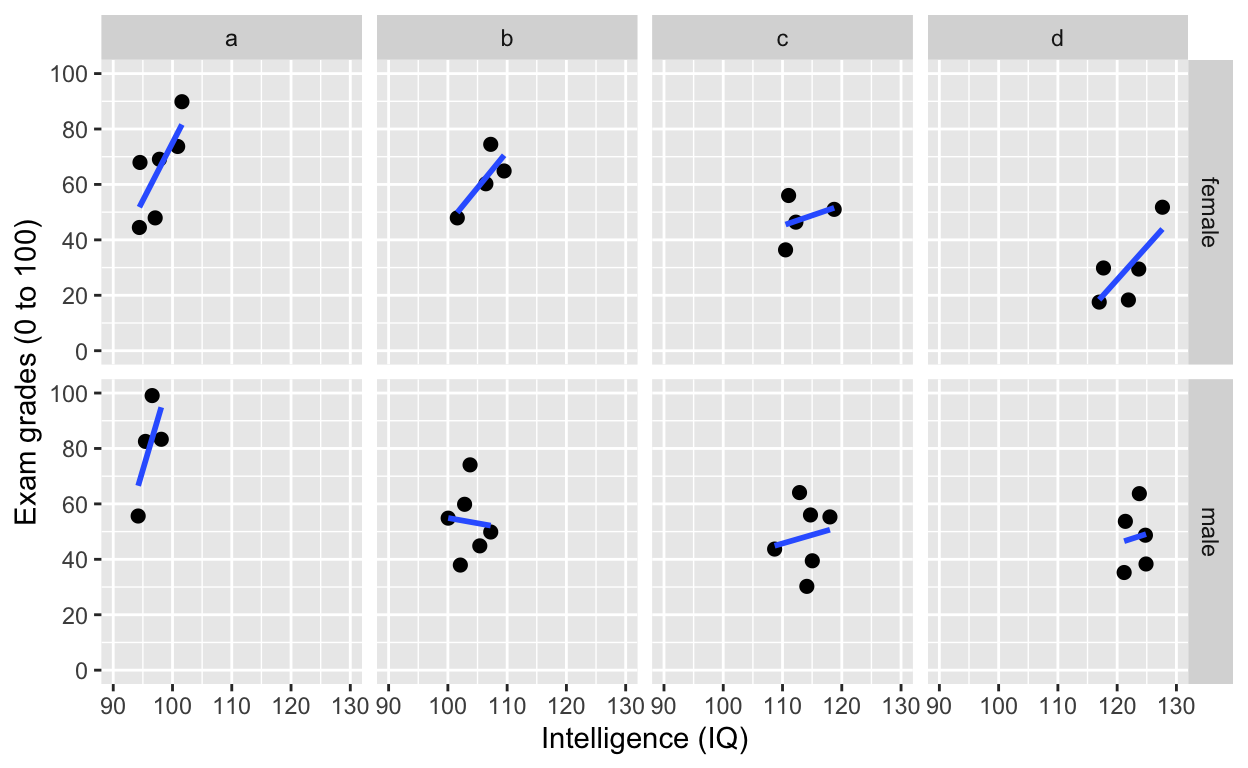`facet_grid()`: one facet per class and gender

``````
geom_point(size = 2) + # change size and colour
labs(y = "Exam grades (0 to 100)", x = "Intelligence (IQ)") + # rename axes
scale_y_continuous(limits = c(0, 100), breaks = c(0, 20, 40, 60, 80, 100)) + # y axis limits/range (0, 100), break points
scale_x_continuous(limits = c(90, 130)) + # x axis limits/range
geom_smooth(method = 'lm', se = F) +
facet_grid(gender~class, labeller = label_both) # one facet per gender``````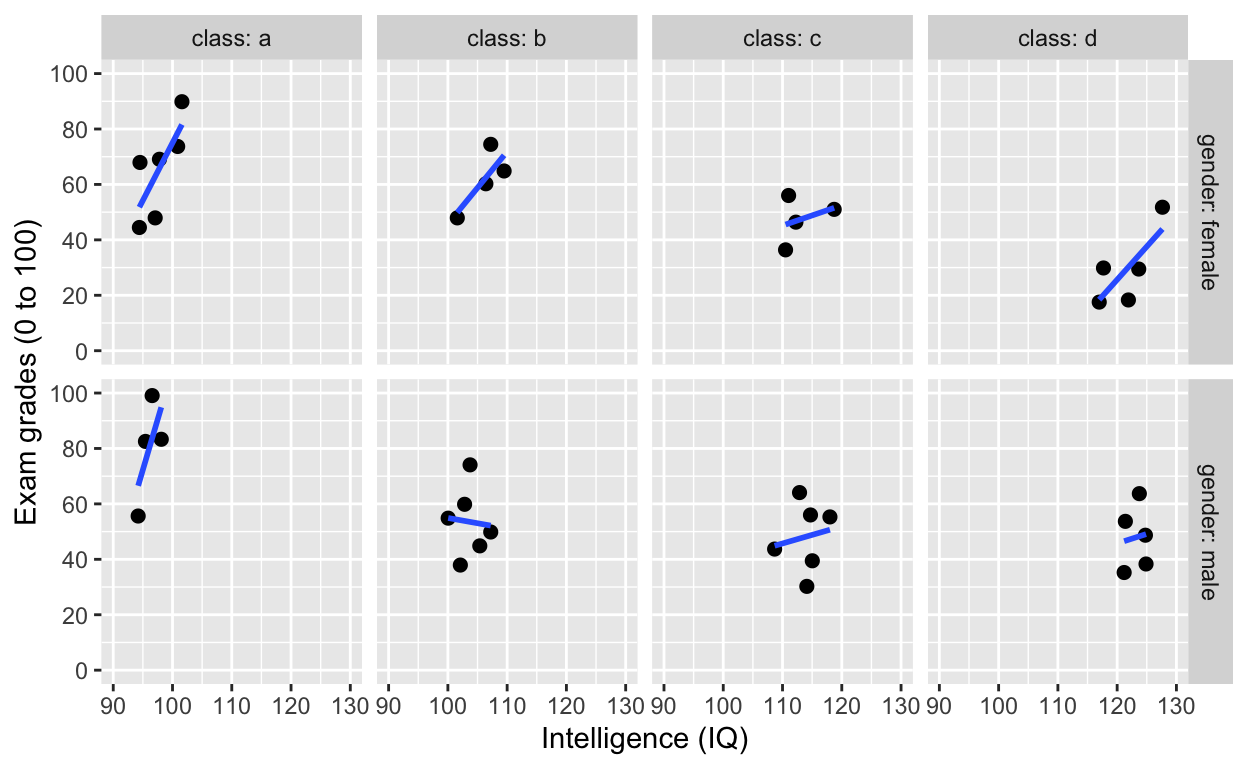## Fitting linear models (general linear model framework)

Fit a model to this this relationship

``````
geom_point() +
labs(y = "Exam grades (0 to 100)", x = "Intelligence (IQ)") + # rename axes
scale_y_continuous(limits = c(0, 100), breaks = c(0, 20, 40, 60, 80, 100)) + # y axis limits/range (0, 100), break points
scale_x_continuous(limits = c(90, 130)) + # x axis limits/range
geom_smooth(method = 'lm', se = F, col = 'black') # fit linear regression line, remove standard error, black line``````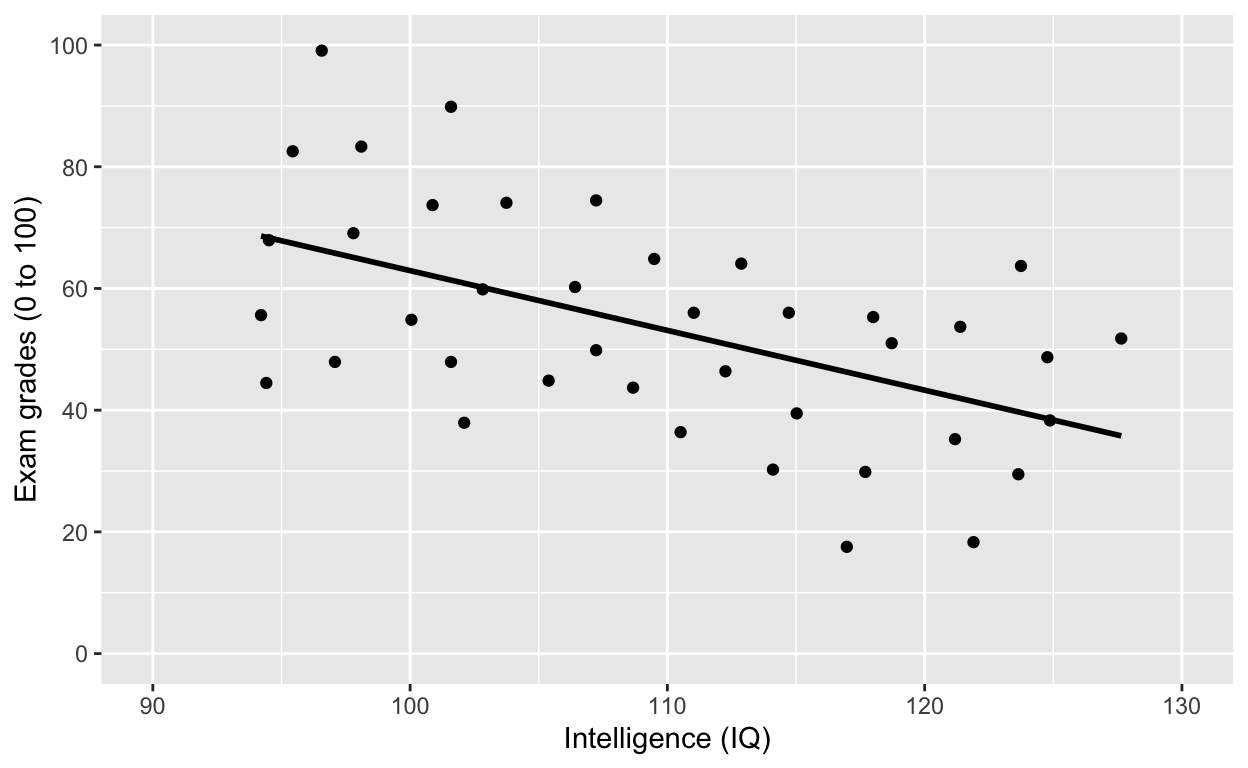### Model specification in R

• most model fitting functions prefer long-form data (aka tidy data)
• ~ is the symbol for “prediction” (read: “predicted by”)
• y ~ x: y predicted by x (y is outcome/dependent variable, x is predictor/independent variable)
• `lm(y ~ x, data)` is the most commonly-used and flexible function (linear model)
• covariates and predictors are specified in the same way (unlike SPSS)

Test the relationship in the plot above

``````
model_linear <- lm(formula = iq ~ grades, data = df1)
summary(model_linear) # get model results and p values``````
``````
Call:
lm(formula = iq ~ grades, data = df1)

Residuals:
Min       1Q   Median       3Q      Max
-17.7002  -4.9650   0.3856   6.0826  17.6721

Coefficients:
Estimate Std. Error t value Pr(>|t|)
(Intercept) 125.14212    4.23923  29.520  < 2e-16 ***
grades       -0.29306    0.07479  -3.919 0.000359 ***
---
Signif. codes:  0 '***' 0.001 '**' 0.01 '*' 0.05 '.' 0.1 ' ' 1

Residual standard error: 8.599 on 38 degrees of freedom
Multiple R-squared:  0.2878,    Adjusted R-squared:  0.2691
F-statistic: 15.36 on 1 and 38 DF,  p-value: 0.0003592``````
``````
summaryh(model_linear) # generates APA-formmatted results (requires hausekeep package)``````
``````
term                                                  results
1: (Intercept) b = 125.14, SE = 4.24, t(38) = 29.52, p < .001, r = 0.98
2:      grades  b = −0.29, SE = 0.07, t(38) = −3.92, p < .001, r = 0.54``````

Note the significant negative relationship between iq and grades.

Since we know that class “moderates” the effect between iq and grades, let’s “control” for class by adding `class` into the model specification.

``````
ggplot(df1, aes(iq, grades, col = class)) +
geom_point(size = 3) + # change size and colour
labs(y = "Exam grades (0 to 100)", x = "Intelligence (IQ)") + # rename axes
scale_y_continuous(limits = c(0, 100), breaks = c(0, 20, 40, 60, 80, 100)) + # y axis limits/range (0, 100), break points
scale_x_continuous(limits = c(90, 130)) + # x axis limits/range
geom_smooth(method = 'lm', se = F) + # fit linear regression line
geom_smooth(method = 'lm', se = F, aes(group = 1))``````Test the relationship above by “controlling” for class

``````
model_linear_class <- lm(iq ~ grades + class, data = df1)
summary(model_linear_class) # get model results and p values``````
``````
Call:
lm(formula = iq ~ grades + class, data = df1)

Residuals:
Min      1Q  Median      3Q     Max
-4.5552 -2.2276 -0.1403  2.0785  4.8499

Coefficients:
Estimate Std. Error t value Pr(>|t|)
(Intercept) 90.74793    2.48240  36.557  < 2e-16 ***
grades       0.08841    0.03251   2.720   0.0101 *
classb       8.82731    1.33606   6.607 1.24e-07 ***
classc      18.61047    1.46538  12.700 1.15e-14 ***
classd      28.21349    1.64119  17.191  < 2e-16 ***
---
Signif. codes:  0 '***' 0.001 '**' 0.01 '*' 0.05 '.' 0.1 ' ' 1

Residual standard error: 2.796 on 35 degrees of freedom
Multiple R-squared:  0.9306,    Adjusted R-squared:  0.9227
F-statistic: 117.4 on 4 and 35 DF,  p-value: < 2.2e-16``````
``````
summaryh(model_linear_class)``````
``````
term                                                 results
1: (Intercept) b = 90.75, SE = 2.48, t(35) = 36.56, p < .001, r = 0.99
2:      grades   b = 0.09, SE = 0.03, t(35) = 2.72, p = .010, r = 0.42
3:      classb   b = 8.83, SE = 1.34, t(35) = 6.61, p < .001, r = 0.74
4:      classc b = 18.61, SE = 1.47, t(35) = 12.70, p < .001, r = 0.91
5:      classd b = 28.21, SE = 1.64, t(35) = 17.19, p < .001, r = 0.95``````

Note the significantly positive relationship between iq and grades now.

### Reference groups and releveling (changing reference group)

R automatically recodes categorical/factor variables into 0s and 1s (i.e., dummy-coding). Alphabets/letters/characters/numbers that come first (a comes before b) will be coded 0, and those that follow will be coded 1.

In our case, class “a” has been coded 0 (reference group) and all other classes (“b”, “c”, “d”) are contrasted against it, hence you have 3 other effects (“classb”, “classc”, “classd”) that reflect the difference between class “a” and each of the other classes.

To change reference group, use `as.factor()` and `relevel()`

To change reference groups, you first have to convert your grouping variable to `factor` class, which explicitly tells R your variable is a categorical/factor variable. Then use `relevel()` to change the reference group.

``````
df1\$class <- relevel(as.factor(df1\$class), ref = "d")
levels(df1\$class) # check reference levels (d is now the reference/first group)``````
``````
 "d" "a" "b" "c"``````
``````
summaryh(lm(iq ~ grades + class, data = df1)) # quickly fit model and look at outcome (no assignment to object)``````
``````
term                                                   results
1: (Intercept)  b = 118.96, SE = 1.54, t(35) = 77.41, p < .001, r = 1.00
2:      grades     b = 0.09, SE = 0.03, t(35) = 2.72, p = .010, r = 0.42
3:      classa b = −28.21, SE = 1.64, t(35) = −17.19, p < .001, r = 0.95
4:      classb b = −19.39, SE = 1.38, t(35) = −14.01, p < .001, r = 0.92
5:      classc   b = −9.60, SE = 1.29, t(35) = −7.47, p < .001, r = 0.78``````

### Specify interactions

• y predicted by x1, x2, and their interactions: y ~ x1 + x2 + x1:x2
• concise expression: y ~ x1 * x2 (includes all main effects and interaction)
``````
model_linear_interact <- lm(iq ~ grades + class + grades:class, data = df1)
summary(model_linear_interact)``````
``````
Call:
lm(formula = iq ~ grades + class + grades:class, data = df1)

Residuals:
Min      1Q  Median      3Q     Max
-4.6623 -2.3238 -0.2229  1.9845  4.9309

Coefficients:
Estimate Std. Error t value Pr(>|t|)
(Intercept)   117.56287    2.57958  45.574  < 2e-16 ***
grades          0.12459    0.06237   1.998  0.05433 .
classa        -25.29661    4.70506  -5.376 6.64e-06 ***
classb        -18.39902    5.29099  -3.477  0.00148 **
classc         -6.97275    5.18349  -1.345  0.18802
---
Signif. codes:  0 '***' 0.001 '**' 0.01 '*' 0.05 '.' 0.1 ' ' 1

Residual standard error: 2.898 on 32 degrees of freedom
Multiple R-squared:  0.9319,    Adjusted R-squared:  0.917
F-statistic: 62.52 on 7 and 32 DF,  p-value: < 2.2e-16``````
``````
summaryh(model_linear_interact)``````
``````
term                                                  results
1:   (Intercept) b = 117.56, SE = 2.58, t(32) = 45.57, p < .001, r = 0.99
2:        grades    b = 0.12, SE = 0.06, t(32) = 2.00, p = .054, r = 0.33
3:        classa b = −25.30, SE = 4.71, t(32) = −5.38, p < .001, r = 0.69
4:        classb b = −18.40, SE = 5.29, t(32) = −3.48, p = .002, r = 0.52
5:        classc  b = −6.97, SE = 5.18, t(32) = −1.35, p = .188, r = 0.23
6: grades:classa  b = −0.06, SE = 0.08, t(32) = −0.70, p = .490, r = 0.12
7: grades:classb  b = −0.03, SE = 0.10, t(32) = −0.29, p = .776, r = 0.05
8: grades:classc  b = −0.06, SE = 0.11, t(32) = −0.56, p = .581, r = 0.10``````

#### Intercept-only model

R uses `1` to refer to the intercept

``````
model_linear_intercept <- lm(iq ~ 1, data = df1) # mean iq
coef(model_linear_intercept) # get coefficients from model``````
``````
(Intercept)
109.4077 ``````
``````
# summaryh(model_linear_intercept)
df1[, mean(iq)] # matches the intercept term``````
``````
 109.4077``````
``````
mean(df1\$iq) # same as above``````
``````
 109.4077``````

Remove intercept from model (if you ever need to do so…) by specifying `-1`. Another way is to specify `0` in the syntax.

``````
model_linear_noIntercept <- lm(iq ~ grades - 1, data = df1) # substract intercept
summary(model_linear_noIntercept)``````
``````
Call:
lm(formula = iq ~ grades - 1, data = df1)

Residuals:
Min      1Q  Median      3Q     Max
-81.586  -6.131  14.935  35.214  88.969

Coefficients:
Estimate Std. Error t value Pr(>|t|)
grades   1.7980     0.1158   15.52   <2e-16 ***
---
Signif. codes:  0 '***' 0.001 '**' 0.01 '*' 0.05 '.' 0.1 ' ' 1

Residual standard error: 41.52 on 39 degrees of freedom
Multiple R-squared:  0.8607,    Adjusted R-squared:  0.8571
F-statistic:   241 on 1 and 39 DF,  p-value: < 2.2e-16``````
``````
# summaryh(model_linear_noIntercept)

coef(lm(iq ~ 0 + grades, data = df1)) # no intercept``````
``````
1.797984 ``````

Be careful when you remove the intercept (or set it to 0). See my article to learn more.

### Fitting ANOVA with `anova` and `aov`

By default, R uses Type I sum of squares.

Let’s test this model with ANOVA.

``````
ggplot(df1, aes(class, iq)) + # y: iq
geom_quasirandom(alpha = 0.3) +
stat_summary(fun = mean, geom = 'point', size = 3) + # apply mean function
stat_summary(fun.data = mean_cl_normal, geom = 'errorbar', width = 0, size = 1) # apply mean_cl_normal function to data``````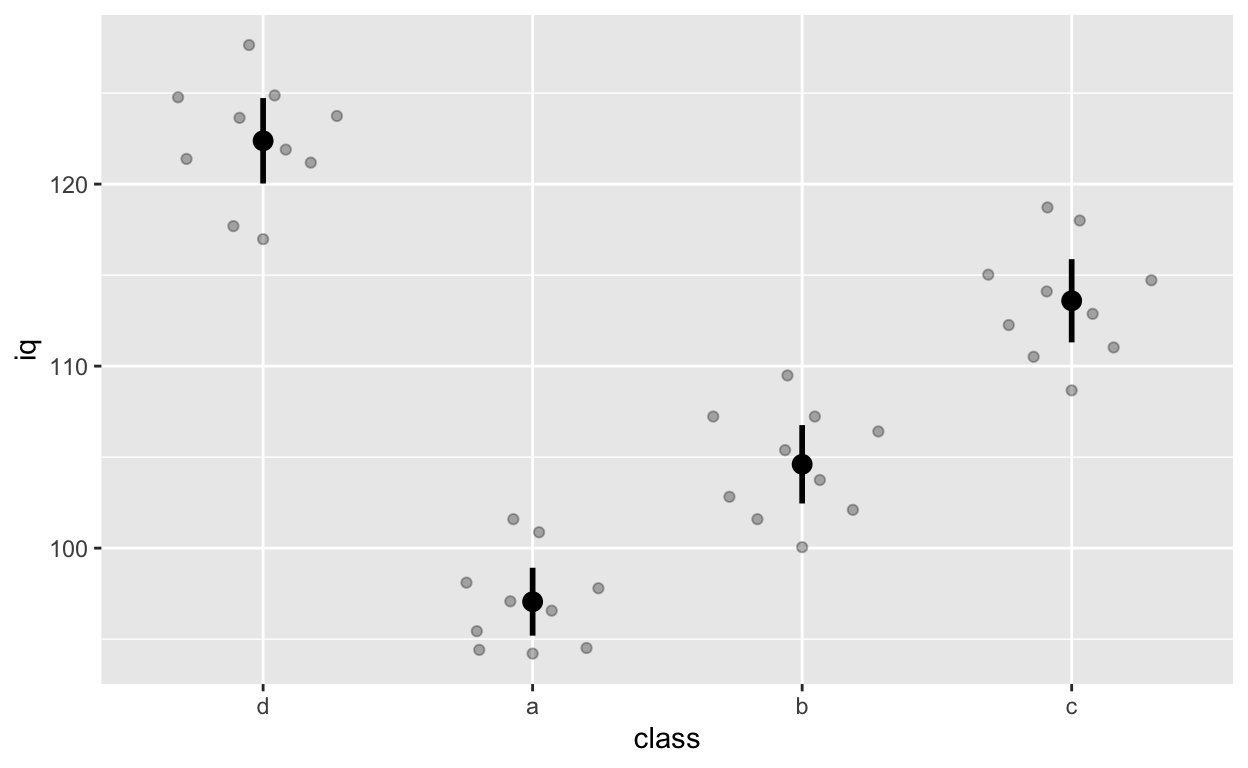Note that class d comes first because we releveled it earlier on (we changed the reference group to d).

Fit ANOVA with `aov()`

``````
anova_class <- aov(grades ~ class, data = df1)
summary(anova_class)``````
``````
Df Sum Sq Mean Sq F value   Pr(>F)
class        3   5821  1940.4    9.44 9.73e-05 ***
Residuals   36   7400   205.6
---
Signif. codes:  0 '***' 0.001 '**' 0.01 '*' 0.05 '.' 0.1 ' ' 1``````

Class * gender interaction (and main effects)

``````
ggplot(df1, aes(class, iq, col = gender)) + # y: iq
geom_quasirandom(alpha = 0.3, dodge = 0.5) +
stat_summary(fun = mean, geom = 'point', size = 3, position = position_dodge(0.5)) +
stat_summary(fun.data = mean_cl_normal, geom = 'errorbar',
width = 0, size = 1, position = position_dodge(0.5))``````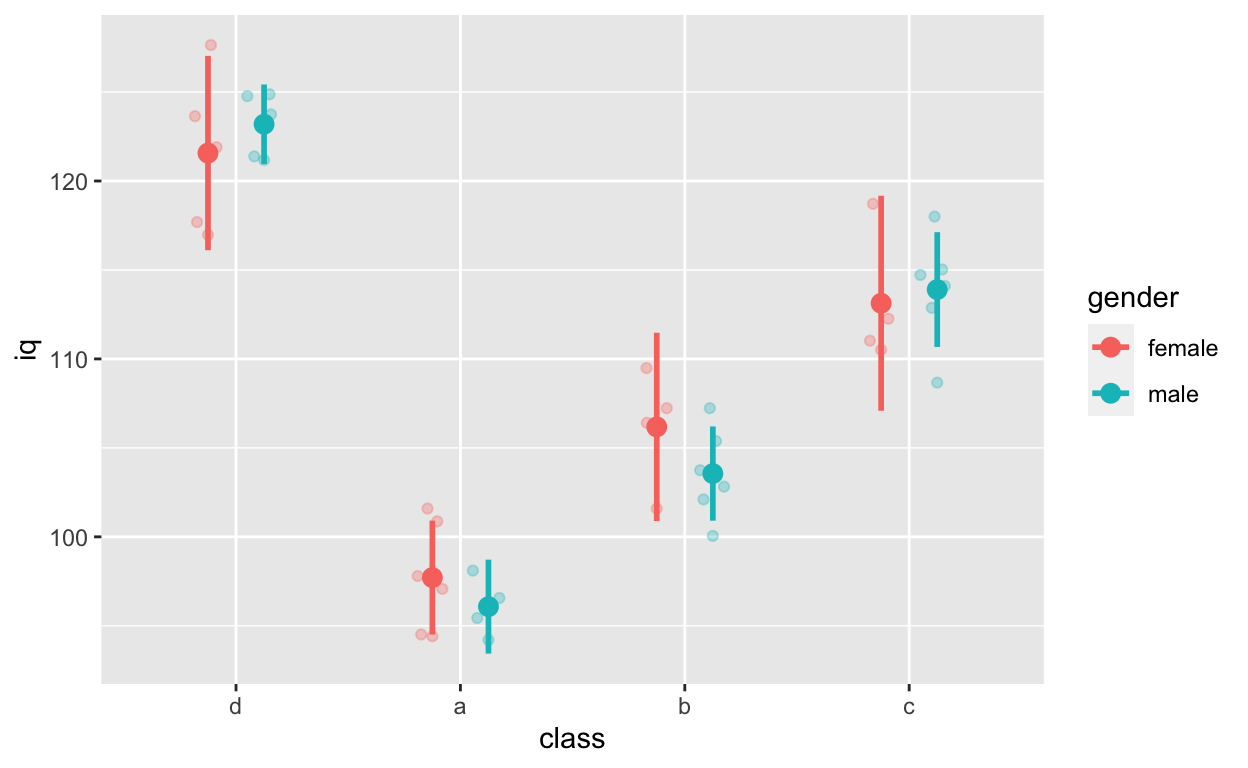``````
anova_classGender <- aov(grades ~ class * gender, data = df1)
anova_classGender``````
``````
Call:
aov(formula = grades ~ class * gender, data = df1)

Terms:
class   gender class:gender Residuals
Sum of Squares  5821.086  412.005     1128.603  5859.355
Deg. of Freedom        3        1            3        32

Residual standard error: 13.53162
Estimated effects may be unbalanced``````

### Fit t-test with `t.test()`

Fit models for this figure

``````
ggplot(df1, aes(class, iq, col = gender)) + # y: iq
geom_quasirandom(alpha = 0.3, dodge = 0.5) +
stat_summary(fun = mean, geom = 'point', size = 3, position = position_dodge(0.5)) +
stat_summary(fun.data = mean_cl_normal, geom = 'errorbar',
width = 0, size = 1, position = position_dodge(0.5))``````Gender effect

``````
ttest_gender <- t.test(iq ~ gender, data = df1)
ttest_gender``````
``````
Welch Two Sample t-test

data:  iq by gender
t = -0.23058, df = 37.645, p-value = 0.8189
alternative hypothesis: true difference in means is not equal to 0
95 percent confidence interval:
-7.269840  5.783542
sample estimates:
mean in group female   mean in group male
109.0175             109.7607 ``````
``````
summaryh(ttest_gender)``````
``````
results
1: t(38) = −0.23, p = .819, r = 0.04``````

class a vs. class d

``````
ttest_classAD <- t.test(iq ~ class, data = df1[class %in% c("a", "d")]) # data.table subsetting
``````
Welch Two Sample t-test

data:  iq by class
t = 19.105, df = 17.128, p-value = 5.488e-13
alternative hypothesis: true difference in means is not equal to 0
95 percent confidence interval:
22.52811 28.11803
sample estimates:
mean in group d mean in group a
122.37948        97.05641 ``````
``````
summaryh(ttest_classAD, showTable = T) # show all other effect sizes``````
``````
\$results
results
1: t(17) = 19.10, p < .001, r = 0.98

\$results2
term     df statistic p.value  es.r  es.d
1: iq by class 17.128    19.105       0 0.977 9.233``````

### Linear mixed effects (aka. multi-level or hierarchical) models with `lmer()` from the `lme4` package

Rather than “control” for class when fitting models to test the relationship between iq and grades below, we can use multi-level models to specify nesting within the data. See here for beautiful visual introduction to multi-level models.

Another function is `nlme()` from the `lme` package. We use both `nlme()` and `lmer()`, depending on our needs.

``````
ggplot(df1, aes(iq, grades, col = class)) +
geom_point(size = 3) + # change size and colour
labs(y = "Exam grades (0 to 100)", x = "Intelligence (IQ)") + # rename axes
scale_y_continuous(limits = c(0, 100), breaks = c(0, 20, 40, 60, 80, 100)) + # y axis limits/range (0, 100), break points
scale_x_continuous(limits = c(90, 130)) + # x axis limits/range
geom_smooth(method = 'lm', se = F) + # fit linear regression line
geom_smooth(method = 'lm', se = F, aes(group = 1))``````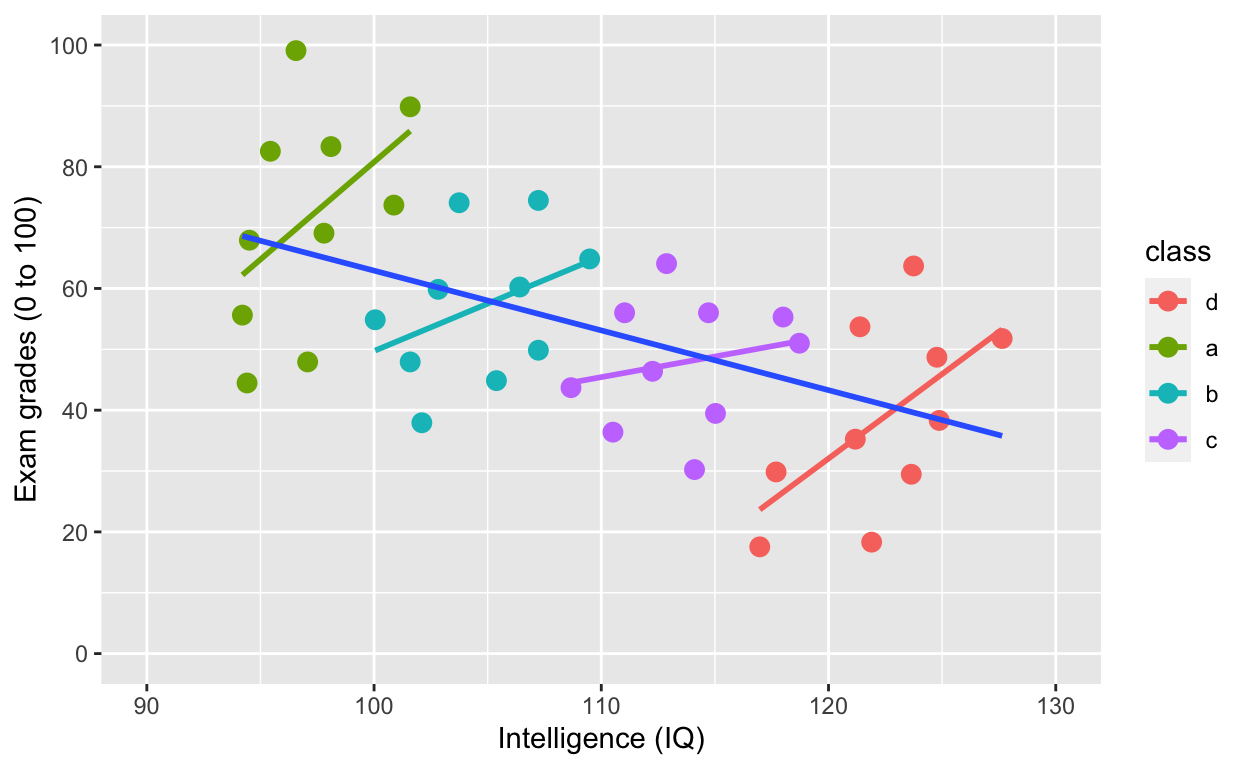Model specification with `lmer()`

• y ~ x (same as other models)
• (1 | group): varying intercept (one intercept per group)
• (1 + x | group): varying intercept and slope (one intercept and slope per group)
• (1 + x || group): varying intercept and slope but no correlation between them

#### Random intercept model (fixed slope)

``````
m_intercept <- lmer(grades ~ iq + (1 | class), data = df1)
summary(m_intercept)``````
``````
Linear mixed model fit by REML. t-tests use Satterthwaite's method [
lmerModLmerTest]
Formula: grades ~ iq + (1 | class)
Data: df1

REML criterion at convergence: 326

Scaled residuals:
Min       1Q   Median       3Q      Max
-1.71341 -0.79563  0.03887  0.56708  2.18978

Random effects:
Groups   Name        Variance Std.Dev.
class    (Intercept) 895.5    29.92
Residual             176.7    13.29
Number of obs: 40, groups:  class, 4

Fixed effects:
Estimate Std. Error        df t value Pr(>|t|)
(Intercept) -100.7338    74.1311   27.4649  -1.359   0.1852
iq             1.4115     0.6633   31.3429   2.128   0.0413 *
---
Signif. codes:  0 '***' 0.001 '**' 0.01 '*' 0.05 '.' 0.1 ' ' 1

Correlation of Fixed Effects:
(Intr)
iq -0.979``````
``````
summaryh(m_intercept)``````
``````
term                                                    results
1: (Intercept) b = −100.73, SE = 74.13, t(27) = −1.36, p = .185, r = 0.25
2:          iq      b = 1.41, SE = 0.66, t(31) = 2.13, p = .041, r = 0.36``````
``````
coef(m_intercept) # check coefficients for each class``````
``````
\$class
(Intercept)       iq
d  -133.42844 1.411459
a   -66.31752 1.411459
b   -90.94789 1.411459
c  -112.24144 1.411459

attr(,"class")
 "coef.mer"``````

By accounting for nesting within class, the relationship between iq and grades is positive!

#### Random intercept and slope model

``````
m_interceptSlope <- lmer(grades ~ iq + (1 + iq | class), data = df1)
summary(m_interceptSlope)``````
``````
Linear mixed model fit by REML. t-tests use Satterthwaite's method [
lmerModLmerTest]
Formula: grades ~ iq + (1 + iq | class)
Data: df1

REML criterion at convergence: 326

Scaled residuals:
Min       1Q   Median       3Q      Max
-1.71457 -0.77510  0.03527  0.57023  2.20255

Random effects:
Groups   Name        Variance  Std.Dev. Corr
class    (Intercept)  51.87048  7.2021
iq            0.04487  0.2118  1.00
Residual             176.37727 13.2807
Number of obs: 40, groups:  class, 4

Fixed effects:
Estimate Std. Error        df t value Pr(>|t|)
(Intercept) -102.2620    72.9396   30.3464  -1.402   0.1711
iq             1.4407     0.6777   11.9275   2.126   0.0551 .
---
Signif. codes:  0 '***' 0.001 '**' 0.01 '*' 0.05 '.' 0.1 ' ' 1

Correlation of Fixed Effects:
(Intr)
iq -0.978
convergence code: 0
boundary (singular) fit: see ?isSingular``````
``````
summaryh(m_interceptSlope)``````
``````
term                                                    results
1: (Intercept) b = −102.26, SE = 72.94, t(30) = −1.40, p = .171, r = 0.25
2:          iq      b = 1.44, SE = 0.68, t(12) = 2.13, p = .055, r = 0.52``````
``````
coef(m_interceptSlope) # check coefficients for each class``````
``````
\$class
(Intercept)       iq
d   -109.8130 1.218571
a    -93.6742 1.693239
b   -100.2293 1.500443
c   -105.3316 1.350377

attr(,"class")
 "coef.mer"``````

#### Random intercept and slope model (no correlations between varying slopes and intercepts)

``````
m_interceptSlope_noCor <- lmer(grades ~ iq + (1 + iq || class), data = df1)
summary(m_interceptSlope_noCor)``````
``````
Linear mixed model fit by REML. t-tests use Satterthwaite's method [
lmerModLmerTest]
Formula: grades ~ iq + (1 + iq || class)
Data: df1

REML criterion at convergence: 326

Scaled residuals:
Min       1Q   Median       3Q      Max
-1.71319 -0.79539  0.03875  0.56712  2.18967

Random effects:
Groups   Name        Variance  Std.Dev.
class    (Intercept) 8.937e+02 29.895487
class.1  iq          1.279e-06  0.001131
Residual             1.767e+02 13.292074
Number of obs: 40, groups:  class, 4

Fixed effects:
Estimate Std. Error        df t value Pr(>|t|)
(Intercept) -100.6290    74.1208   27.4574  -1.358   0.1856
iq             1.4105     0.6633   31.3229   2.127   0.0414 *
---
Signif. codes:  0 '***' 0.001 '**' 0.01 '*' 0.05 '.' 0.1 ' ' 1

Correlation of Fixed Effects:
(Intr)
iq -0.979
convergence code: 0
boundary (singular) fit: see ?isSingular``````
``````
summaryh(m_interceptSlope_noCor)``````
``````
term                                                    results
1: (Intercept) b = −100.63, SE = 74.12, t(27) = −1.36, p = .186, r = 0.25
2:          iq      b = 1.41, SE = 0.66, t(31) = 2.13, p = .041, r = 0.36``````
``````
coef(m_interceptSlope_noCor) # check coefficients for each class``````
``````
\$class
(Intercept)       iq
d   -133.3096 1.410497
a    -66.2263 1.410507
b    -90.8482 1.410503
c   -112.1320 1.410499

attr(,"class")
 "coef.mer"``````

#### Random slope model (fixed intercept)

``````
m_slope <- lmer(grades ~ iq + (0 + iq | class), data = df1)
summary(m_slope)``````
``````
Linear mixed model fit by REML. t-tests use Satterthwaite's method [
lmerModLmerTest]
Formula: grades ~ iq + (0 + iq | class)
Data: df1

REML criterion at convergence: 326

Scaled residuals:
Min       1Q   Median       3Q      Max
-1.71468 -0.75940  0.02992  0.57630  2.20673

Random effects:
Groups   Name Variance  Std.Dev.
class    iq     0.07826  0.2797
Residual      176.30897 13.2781
Number of obs: 40, groups:  class, 4

Fixed effects:
Estimate Std. Error        df t value Pr(>|t|)
(Intercept) -102.5810    72.8999   31.8200  -1.407   0.1691
iq             1.4484     0.6854   27.8157   2.113   0.0437 *
---
Signif. codes:  0 '***' 0.001 '**' 0.01 '*' 0.05 '.' 0.1 ' ' 1

Correlation of Fixed Effects:
(Intr)
iq -0.979``````
``````
summaryh(m_slope)``````
``````
term                                                    results
1: (Intercept) b = −102.58, SE = 72.90, t(32) = −1.41, p = .169, r = 0.24
2:          iq      b = 1.45, SE = 0.69, t(28) = 2.11, p = .044, r = 0.37``````
``````
coef(m_slope) # check coefficients for each class``````
``````
\$class
(Intercept)       iq
d    -102.581 1.159517
a    -102.581 1.784959
b    -102.581 1.523003
c    -102.581 1.326087

attr(,"class")
 "coef.mer"``````

## MANOVA

Let’s use a different dataset. `iris`, a famous dataset that comes with R. Type `?iris` in your console for more information about this dataset.

``````
irisDT <- as.data.table(iris) # convert to data.table and tibble
irisDT # wide form data``````
``````
Sepal.Length Sepal.Width Petal.Length Petal.Width   Species
1:          5.1         3.5          1.4         0.2    setosa
2:          4.9         3.0          1.4         0.2    setosa
3:          4.7         3.2          1.3         0.2    setosa
4:          4.6         3.1          1.5         0.2    setosa
5:          5.0         3.6          1.4         0.2    setosa
---
146:          6.7         3.0          5.2         2.3 virginica
147:          6.3         2.5          5.0         1.9 virginica
148:          6.5         3.0          5.2         2.0 virginica
149:          6.2         3.4          5.4         2.3 virginica
150:          5.9         3.0          5.1         1.8 virginica``````

The dataset is in wide form. To visualize easily with `ggplot`, we need to convert it to long form (more on converting between forms) in future tutorials.

``````
gather(irisDT, meaureLength, length, -Species) %>% # convert from wide to long form
ggplot(aes(Species, length, col = meaureLength)) + # no need to specify data because of piping
geom_quasirandom(alpha = 0.3, dodge = 0.5) ``````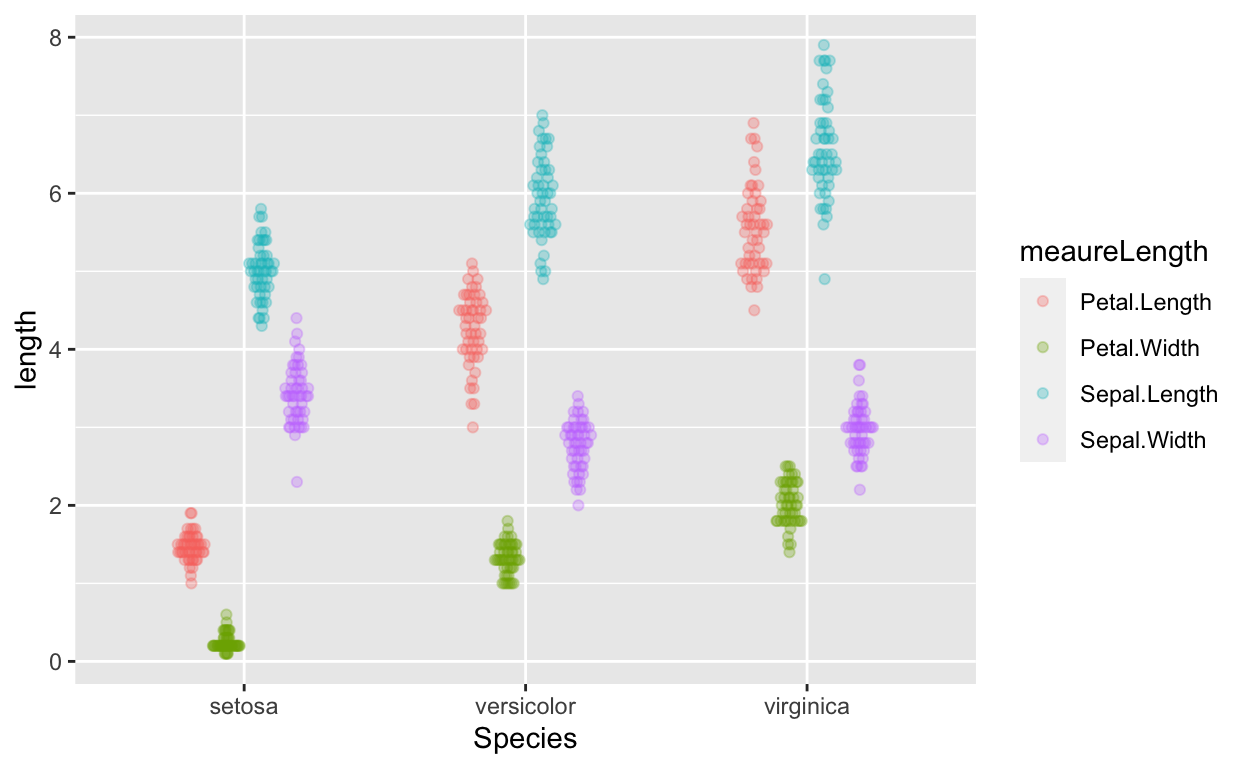MANOVA to test if species predicts length of sepal length and petal length?

``````
outcome <- cbind(irisDT\$Sepal.Length, irisDT\$Petal.Length) # cbind (column bind)
manova_results <- manova(outcome ~ Species, data = iris)
summary(manova_results) # manova results``````
``````
Df Pillai approx F num Df den Df    Pr(>F)
Species     2 0.9885   71.829      4    294 < 2.2e-16 ***
Residuals 147
---
Signif. codes:  0 '***' 0.001 '**' 0.01 '*' 0.05 '.' 0.1 ' ' 1``````
``````
summary.aov(manova_results) # see which outcome variables differ``````
``````
Response 1 :
Df Sum Sq Mean Sq F value    Pr(>F)
Species       2 63.212  31.606  119.26 < 2.2e-16 ***
Residuals   147 38.956   0.265
---
Signif. codes:  0 '***' 0.001 '**' 0.01 '*' 0.05 '.' 0.1 ' ' 1

Response 2 :
Df Sum Sq Mean Sq F value    Pr(>F)
Species       2 437.10 218.551  1180.2 < 2.2e-16 ***
Residuals   147  27.22   0.185
---
Signif. codes:  0 '***' 0.001 '**' 0.01 '*' 0.05 '.' 0.1 ' ' 1``````

### Computing between- and within-subjects error bars (also between-within designs)

Error bars for between- and within-subjects designs have to be calculated differently. There’s much debate on how to compute within-subjects this properly…

``````
cw <- as.data.table(ChickWeight) # convert built-in ChickWeight data to data.table and tibble``````

data information

• ID variable: Chick (50 chicks)
• outcome/dependent variable: weight (weight of Chick) (within-subjects variable)
• predictor/indepedent variable: Diet (diet each Chick was assigned to) (between-subjects variable)
``````
cw # weight of 50 chicks are different times, on different diets``````
``````
weight Time Chick Diet
1:     42    0     1    1
2:     51    2     1    1
3:     59    4     1    1
4:     64    6     1    1
5:     76    8     1    1
---
574:    175   14    50    4
575:    205   16    50    4
576:    234   18    50    4
577:    264   20    50    4
578:    264   21    50    4``````
``````
cw[, unique(Time)] # time points``````
``````
  0  2  4  6  8 10 12 14 16 18 20 21``````
``````
cw[, n_distinct(Chick)] # no. of Chicks``````
``````
 50``````
``````
cw[, unique(Diet)] # Diets``````
``````
 1 2 3 4
Levels: 1 2 3 4``````

#### Between-subject error bars

Do different diets lead to different weights? Each chick is only assigned to one diet (rather than > 1 diet), so we can use between-subjects error bars (or confidence intervals).

``````
ggplot(cw, aes(Diet, weight)) +
geom_quasirandom(alpha = 0.3) + # this line plots raw data and can be omitted, depending on your plotting preferences
stat_summary(fun = mean, geom = 'point', size = 5) + # compute mean and plot
stat_summary(fun.data = mean_cl_normal, geom = 'errorbar', width = 0, size = 1) # compute between-sub confidence intervals``````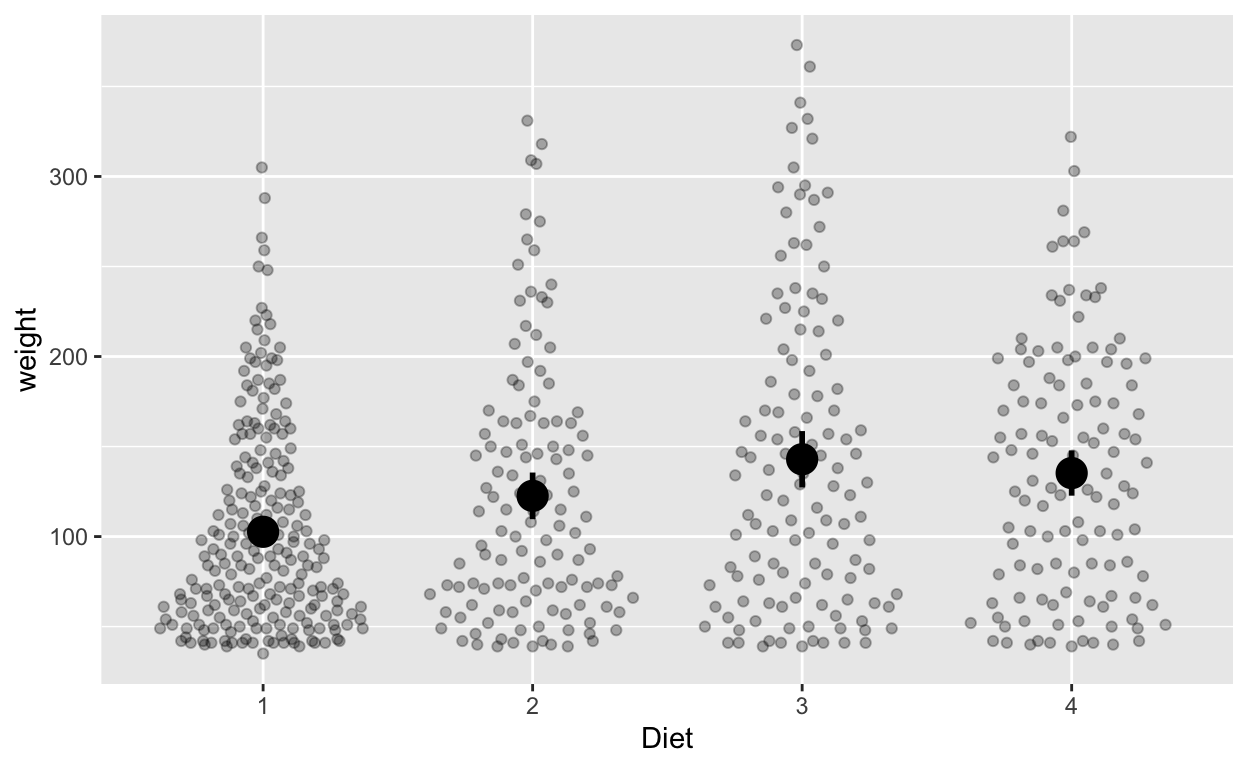#### Within-subject error bars

How does weight change over time (ignoring diet)? Each chick has multiple measurements of time, so we’ll use within-subjects error bars, which we have to calculate ourselves. Use `seWithin()` from the `hausekeep` package to compute within-subjects error bars.

``````
cw_weight_withinEB <- seWithin(data = cw, measurevar = c("weight"),
withinvars = c("Time"), idvar = "Chick")``````

The output contains the mean weight at each time, number of values (N), standard deviation, standard error, and confidence interval (default 95% unless you change via the `conf.interval` argument). The output contains information you’ll use for plotting with `ggplot`.

Plot with within-subjects error bars

``````
ggplot(cw_weight_withinEB, aes(Time, weight)) +
geom_quasirandom(data = cw, alpha = 0.1) + # this line plots raw data and can be omitted, depending on your plotting
geom_errorbar(aes(ymin = weight - ci, ymax = weight + ci), width = 0) # ymin (lower bound), ymax (upper bound)``````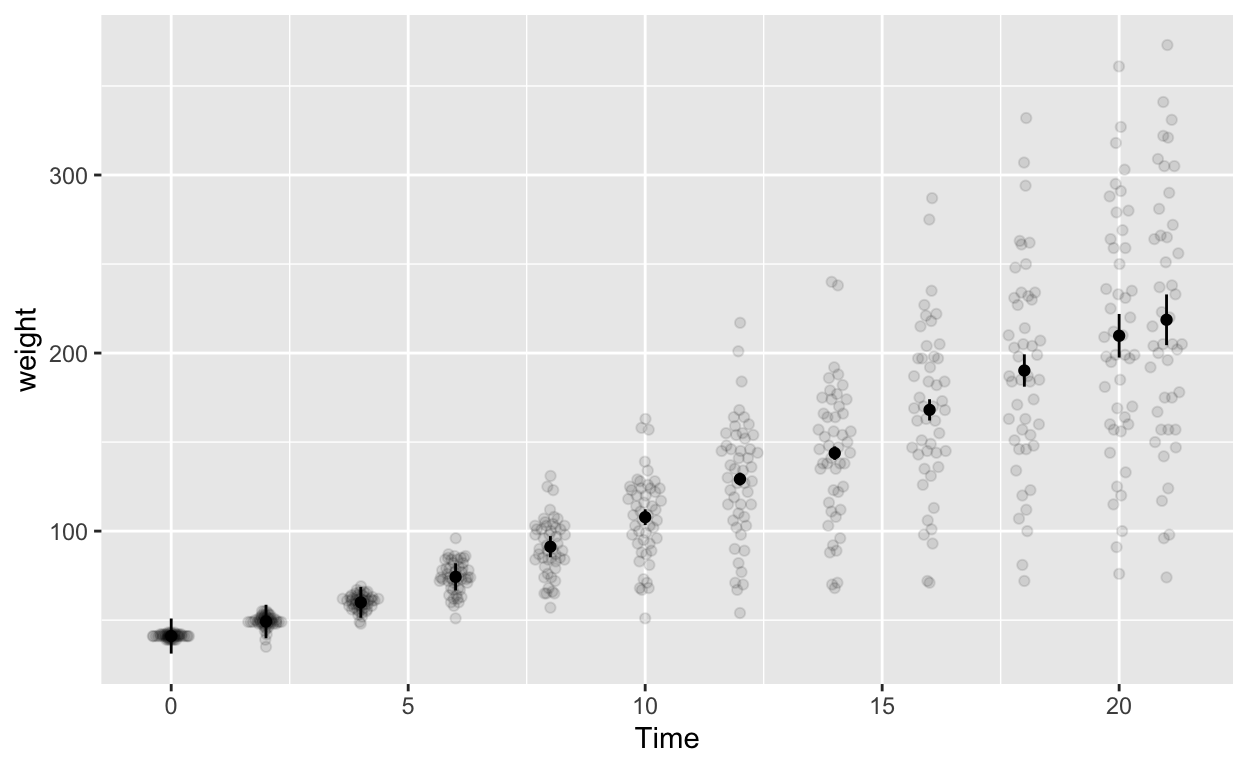Note the second line `geom_quasirandom(data = cw, alpha = 0.1)` adds the raw data to the plot (hence `data = cw`). Depending your data structure and research questions, you might have to compute your “raw data” for the plot differently before specifying it in `geom_quasirandom()`.

Plot with between-subjects error bars (WRONG but illustrative purposes)

``````
ggplot(cw, aes(Time, weight)) +
geom_quasirandom(alpha = 0.1) + # this line plots raw data and can be omitted, depending on your plotting preferences
stat_summary(fun = mean, geom = 'point') + # compute mean and plot
stat_summary(fun.data = mean_cl_normal, geom = 'errorbar', width = 0) # compute between-sub confidence intervals``````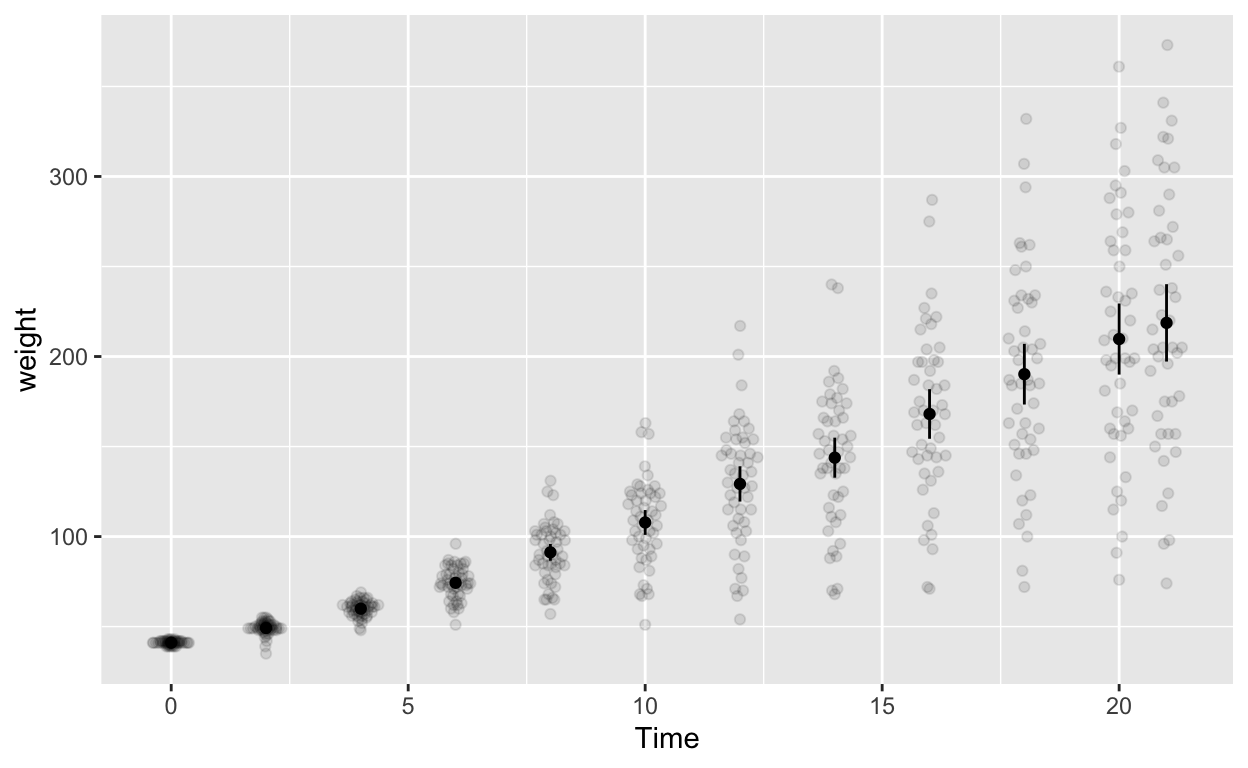#### Mixed (between-within) designs

Let’s investigate the effects of time (within-subjects) and diet (between-subjects) together.

``````
cw_weight_mixed <- seWithin(data = cw, measurevar = c("weight"),
betweenvars = c("Diet"), withinvars = c("Time"),
idvar = "Chick")``````

Now your summary output has the `Diet` column.

``````
ggplot(cw_weight_mixed, aes(Time, weight, col = as.factor(Diet))) + # Diet is numeric but we want it to be a factor/categorical variable
geom_quasirandom(data = cw, alpha = 0.3, dodge = 0.7) + # this line plots raw data and can be omitted, depending on your plotting
geom_point(position = position_dodge(0.7), size = 2.5) + # add points
geom_errorbar(aes(ymin = weight - ci, ymax = weight + ci), width = 0, position = position_dodge(0.7), size = 1) + # ymin (lower bound), ymax (upper bound)
labs(col = "Diet")``````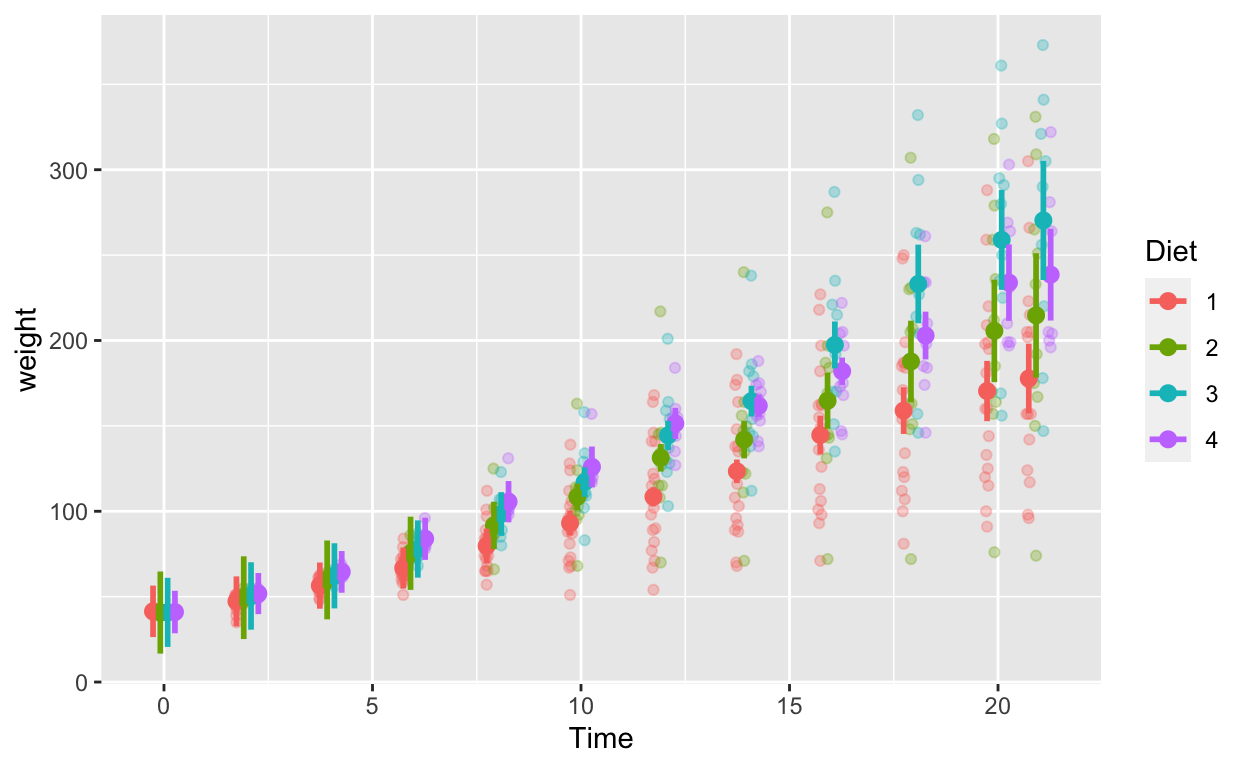## Support my work

### Corrections

If you see mistakes or want to suggest changes, please create an issue on the source repository.

### Reuse

Text and figures are licensed under Creative Commons Attribution CC BY 4.0. Source code is available at https://github.com/hauselin/rtutorialsite, unless otherwise noted. The figures that have been reused from other sources don't fall under this license and can be recognized by a note in their caption: "Figure from ...".